The analysis functions in MassWateR can be used to evaluate trends, summaries, and maps once the required data are successfully imported into R (see the data input and checks vignette for an overview). The results data file with the monitoring data is required. The data quality objectives file for accuracy is also required to automatically determine plot axis scaling as arithmetic (linear) or logarithmic and to fill results data that are below detection or above quantitation limits. The site metadata file is used to create maps. The example data included with the package are imported here to demonstrate how to use the analysis functions:

library(MassWateR)

# import results data
respth <- system.file("extdata/ExampleResults.xlsx", package = "MassWateR")
#> Running checks on results data...
#>  Checking column names... OK
#>  Checking all required columns are present... OK
#>  Checking valid Activity Types... OK
#>  Checking Activity Start Date formats... OK
#>  Checking depth data present... OK
#>  Checking for non-numeric values in Activity Depth/Height Measure... OK
#>  Checking Activity Depth/Height Unit... OK
#>  Checking Activity Relative Depth Name formats... OK
#>  Checking values in Activity Depth/Height Measure > 1 m / 3.3 ft... OK
#>  Checking Characteristic Name formats... OK
#>  Checking Result Values... OK
#>  Checking QC Reference Values... OK
#>  Checking for missing entries for Result Unit... OK
#>  Checking if more than one unit per Characteristic Name... OK
#>  Checking acceptable units for each entry in Characteristic Name... OK
#>
#> All checks passed!

# import accuracy data
accpth <- system.file("extdata/ExampleDQOAccuracy.xlsx", package = "MassWateR")
#> Running checks on data quality objectives for accuracy...
#>  Checking column names... OK
#>  Checking all required columns are present... OK
#>  Checking column types... OK
#>  Checking no "na" in Value Range... OK
#>  Checking for text other than <=, ≤, <, >=, ≥, >, ±, %, AQL, BQL, log, or all... OK
#>  Checking number of rows per parameter... OK
#>  Checking overlaps in Value Range... OK
#>  Checking gaps in Value Range... OK
#>  Checking Parameter formats... OK
#>  Checking for missing entries for unit (uom)... OK
#>  Checking if more than one unit (uom) per Parameter... OK
#>  Checking acceptable units (uom) for each entry in Parameter... OK
#>  Checking empty columns... OK
#>
#> All checks passed!

sitpth <- system.file("extdata/ExampleSites.xlsx", package = "MassWateR")
#> Running checks on site metadata...
#>  Checking column names... OK
#>  Checking all required columns are present... OK
#>  Checking for missing latitude or longitude values... OK
#>  Checking for non-numeric values in latitude... OK
#>  Checking for non-numeric values in longitude... OK
#>  Checking for positive values in longitude... OK
#>  Checking for missing entries for Monitoring Location ID... OK
#>
#> All checks passed!

Seasonal trends can be evaluated using the anlzMWRseason() function. This function summarizes results for a single parameter using boxplots or barplots with seasonal groups assigned to months or weeks of the year. Boxplots or barplots can also include jittered points of the observations on top or only the jittered points can be shown. Threshold lines are also shown on the plot to determine if results are near thresholds of interest. The data that are plotted can be filtered by sites, dates, result attributes, or location groups (from the site metadata file).

The anlzMWRseason() function uses the results data as a file path or data frame as input. The input files are passed to the function using the res and acc arguments, but a named list with the same arguments can also be used with the fset argument for convenience. Below, the results for dissolved oxygen (param = "DO") are grouped by month using group = "month" and as boxplots using type = "box". A description of the summary statistics shown by a boxplot is provided in the outlier checks vignette. The relevant threshold lines for freshwater environments are shown using thresh = "fresh".

anlzMWRseason(res = resdat, param = "DO", acc = accdat, thresh = "fresh", group = "month", type = "box")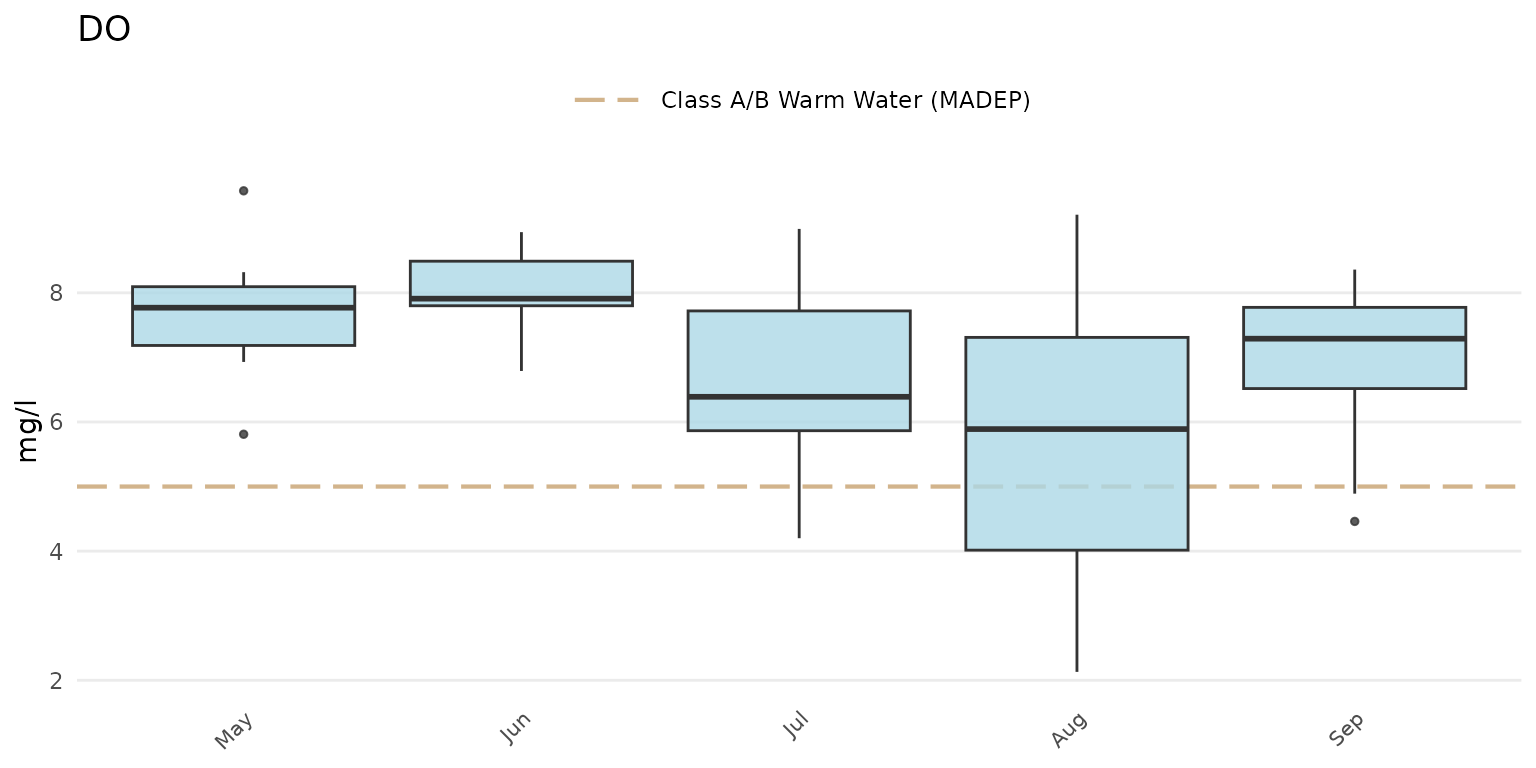Jittered points over the boxplots can be shown by setting type = "jitterbox".

anlzMWRseason(res = resdat, param = "DO", acc = accdat, thresh = "fresh", group = "month", type = "jitterbox")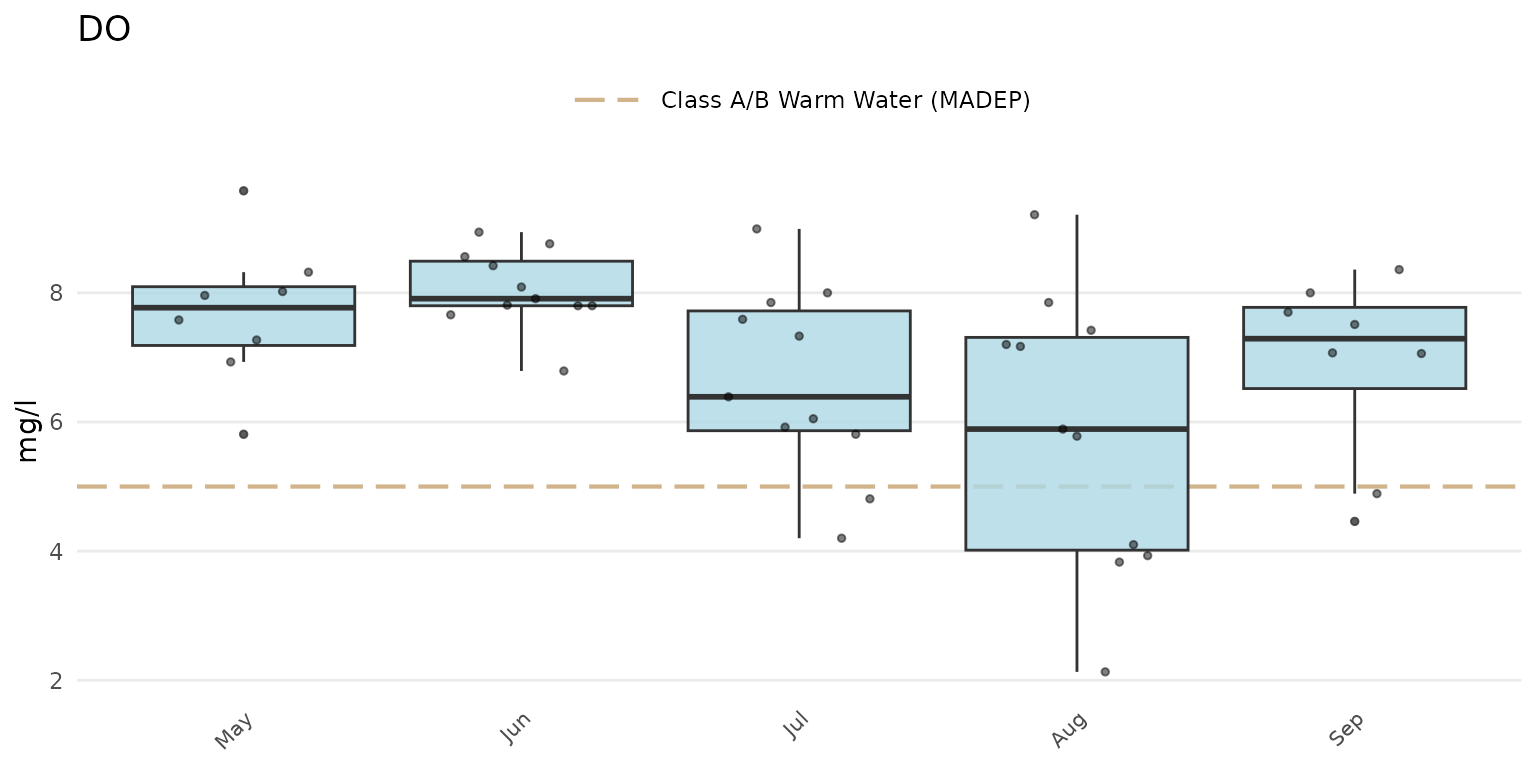The same data can also be grouped by week using group = "week". The week of the year is shown on the plot as an integer. Note that there can be no common month/day indicating the start of the week between years and an integer is the only way to compare summaries if the results data span multiple years.

anlzMWRseason(res = resdat, param = "DO", acc = accdat, thresh = "fresh", group = "week", type = "box")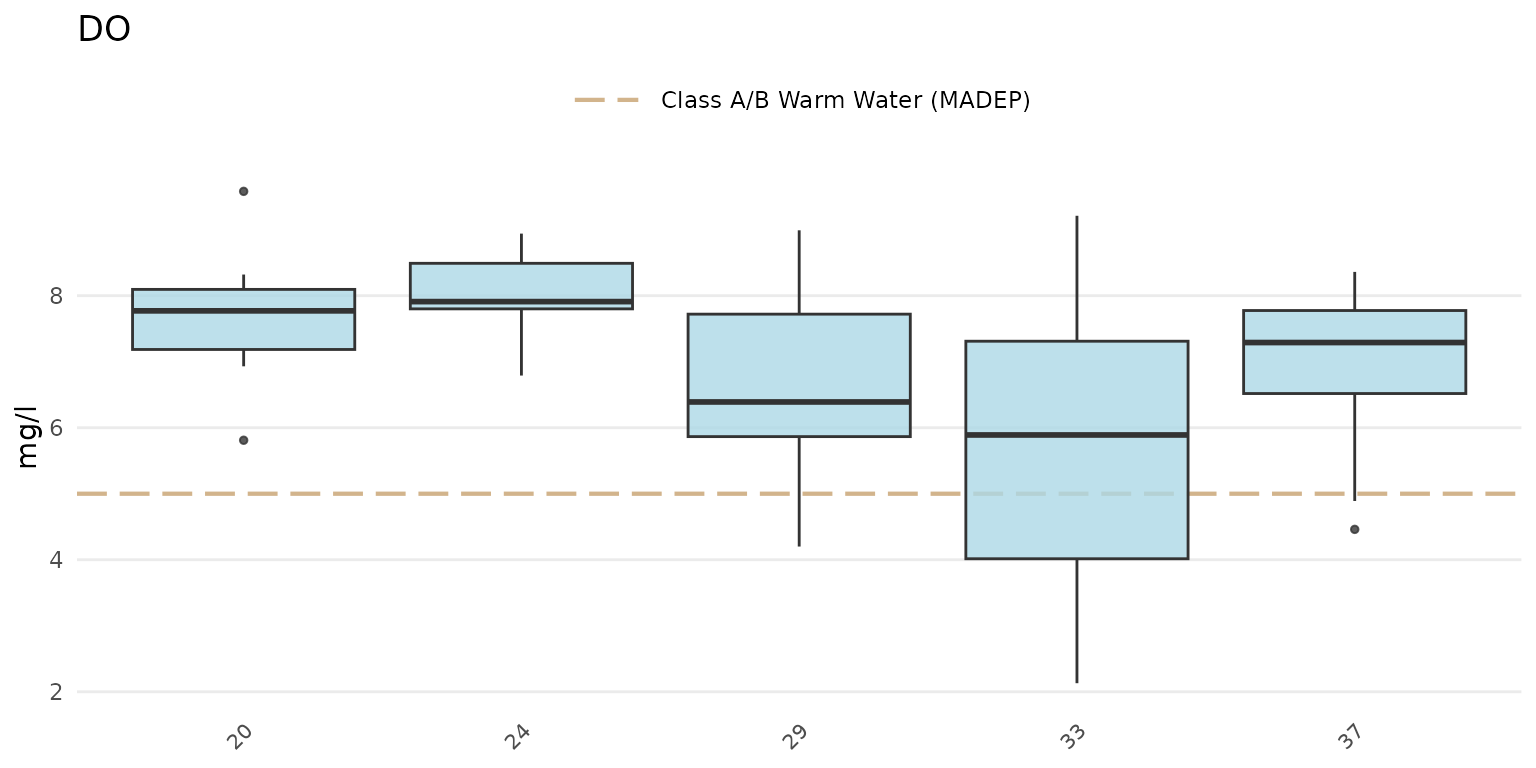Barplots can be shown using type = "bar". The barplots show the average estimate for the results defined by the group argument.

anlzMWRseason(res = resdat, param = "DO", acc = accdat, thresh = "fresh", group = "month", type = "bar")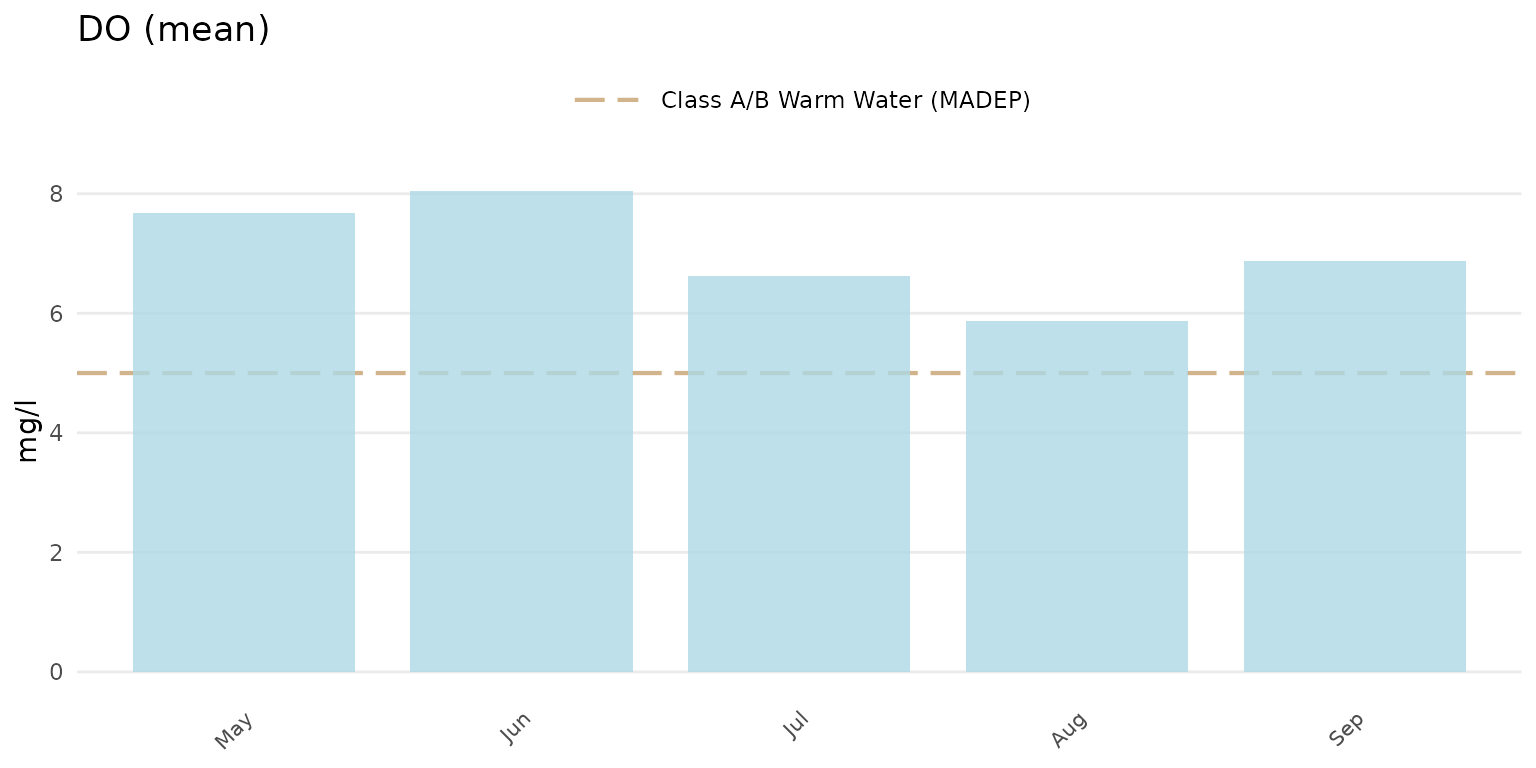Confidence intervals at 95% for the barplots can be shown by setting confint = TRUE.

anlzMWRseason(res = resdat, param = "DO", acc = accdat, thresh = "fresh", group = "month", type = "bar", confint = TRUE)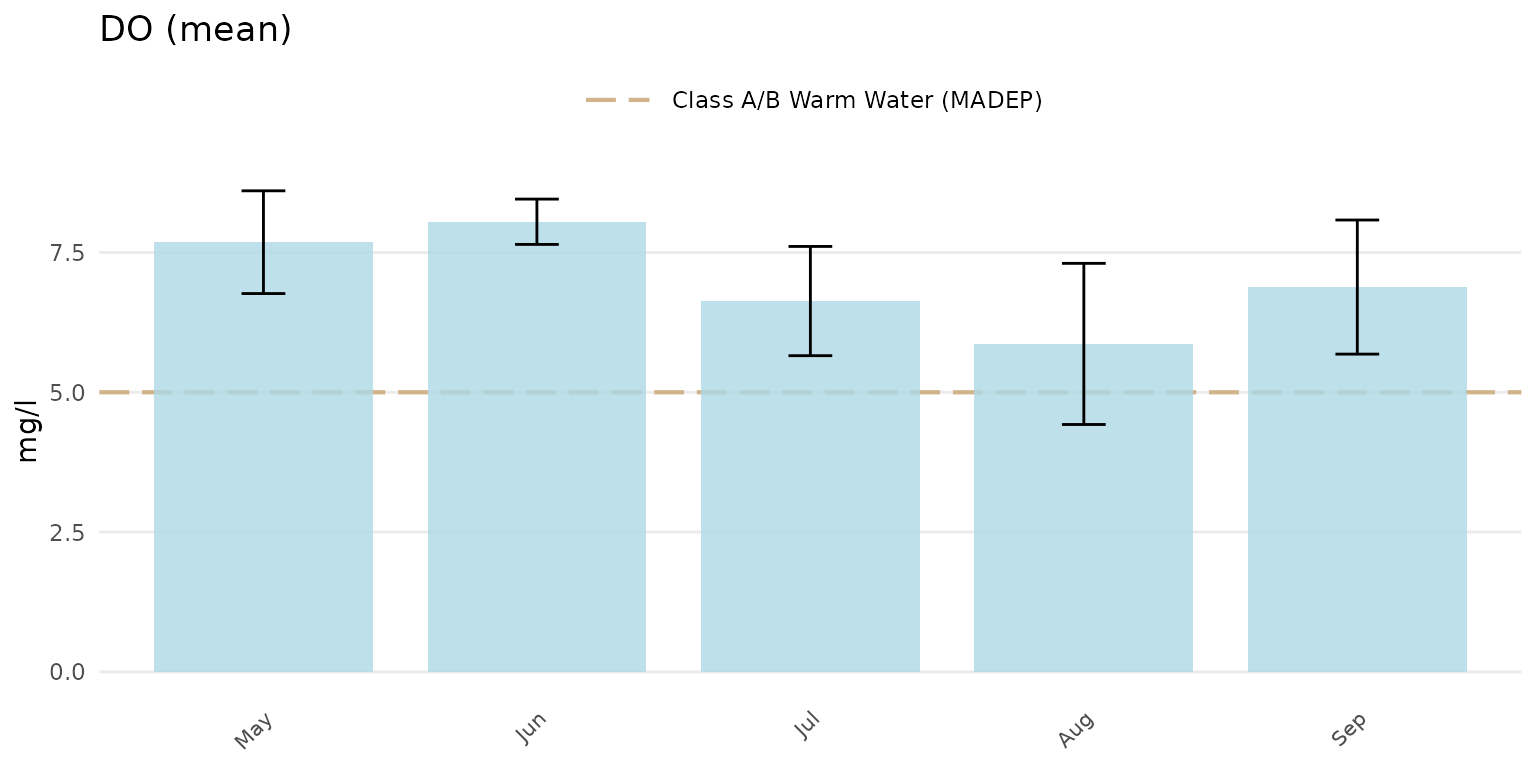Jittered points over the barplots can be shown by setting type = "jitterbar".

anlzMWRseason(res = resdat, param = "DO", acc = accdat, thresh = "fresh", group = "month", type = "jitterbar")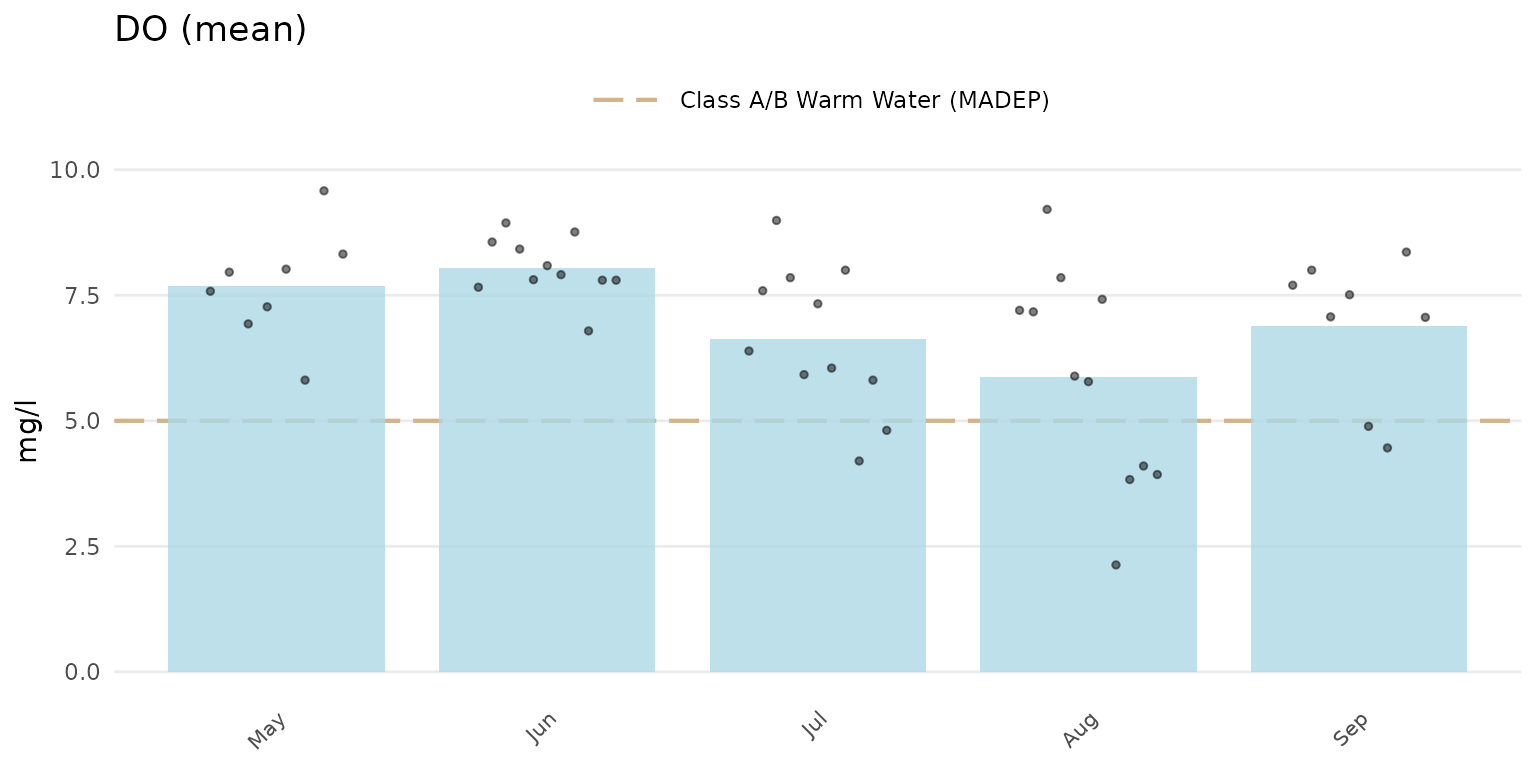Setting type = "jitter" will show only the jittered points.

anlzMWRseason(res = resdat, param = "DO", acc = accdat, thresh = "fresh", group = "month", type = "jitter")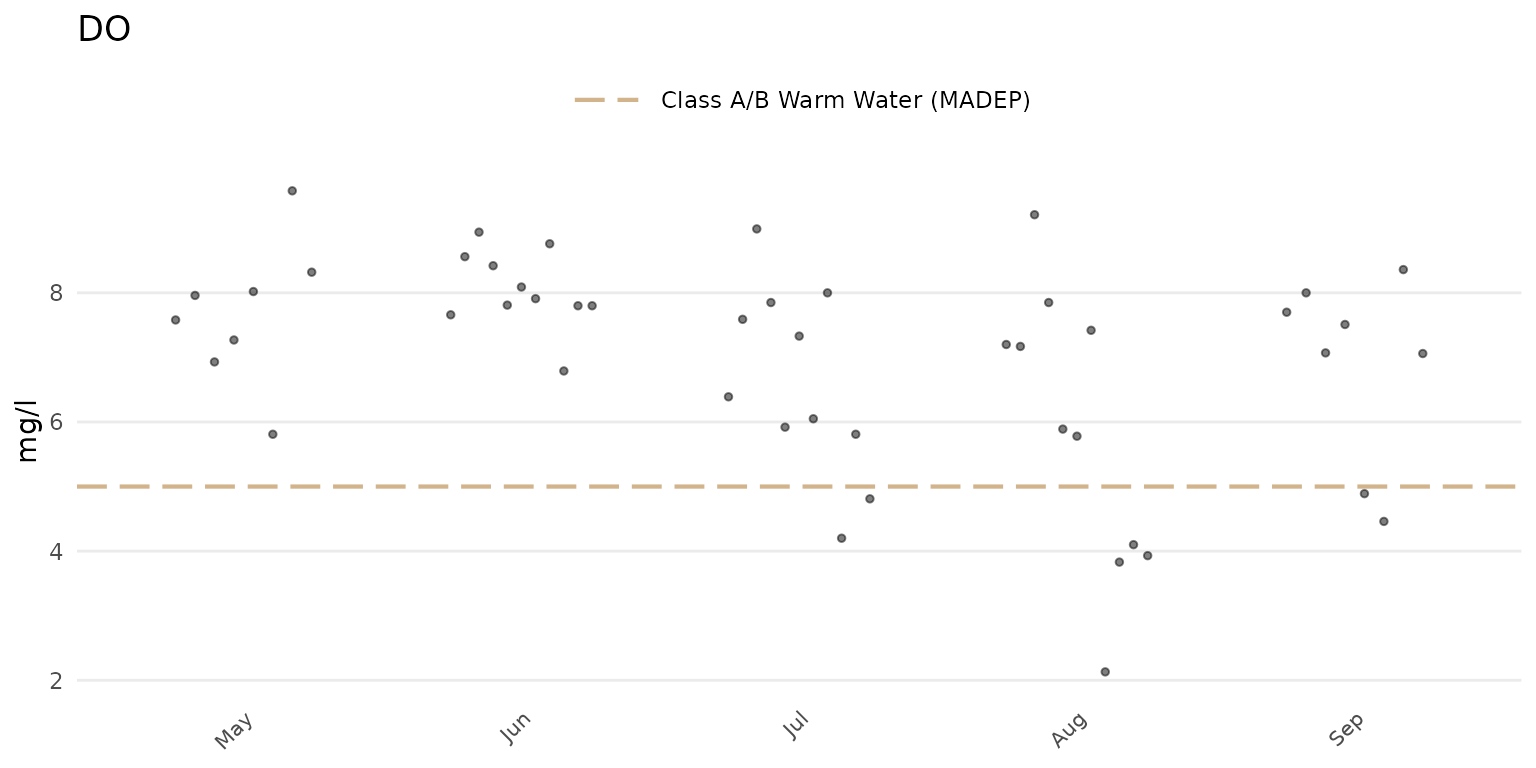The threshold lines shown on each plot are determined from the thresholdMWR file included with MassWateR. These threshold lines describe relevant state standards or typical ranges in Massachusetts, if applicable for a parameter. Thresholds can be plotted for freshwater or marine environments using the thresh argument as thresh = "fresh" or thresh = "marine", respectively. Two threshold lines are typically shown, whereas some parameters may only have one threshold line. Other parameters have no information in the thresholdMWR file and lines will not be plotted. Threshold lines can be suppressed by setting thresh = "none". Below, the marine thresholds are shown for dissolved oxygen using the barplot option grouped by month.

anlzMWRseason(res = resdat, param = "DO", acc = accdat, thresh = "marine", group = "month", type = "bar")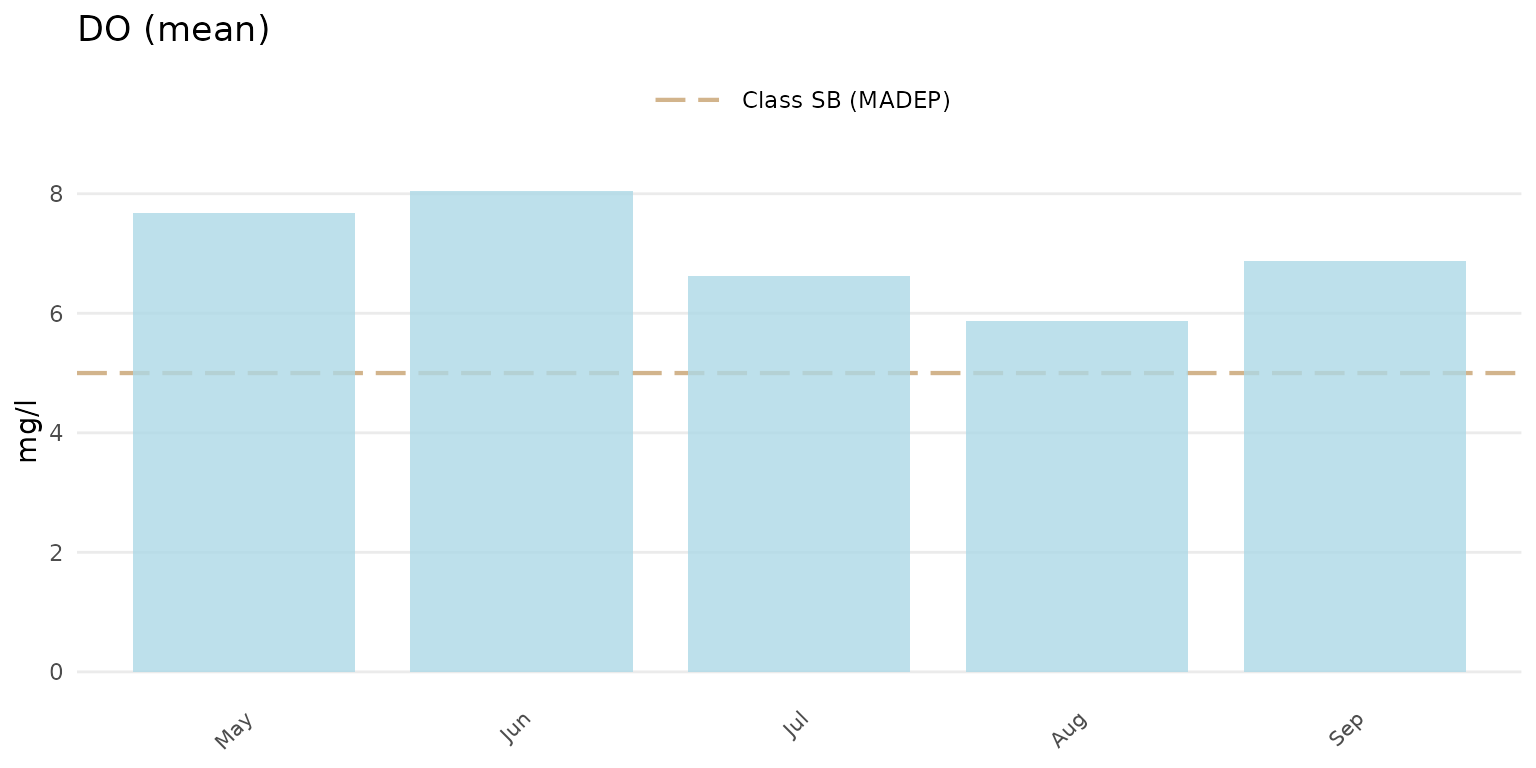A user-supplied numeric value can also be used for the thresh argument. The threshlab argument must also be used to label the custom threshold in the plot legend.

anlzMWRseason(res = resdat, param = "DO", acc = accdat, thresh = 8, threshlab = "Custom threshold", group = "month", type = "bar")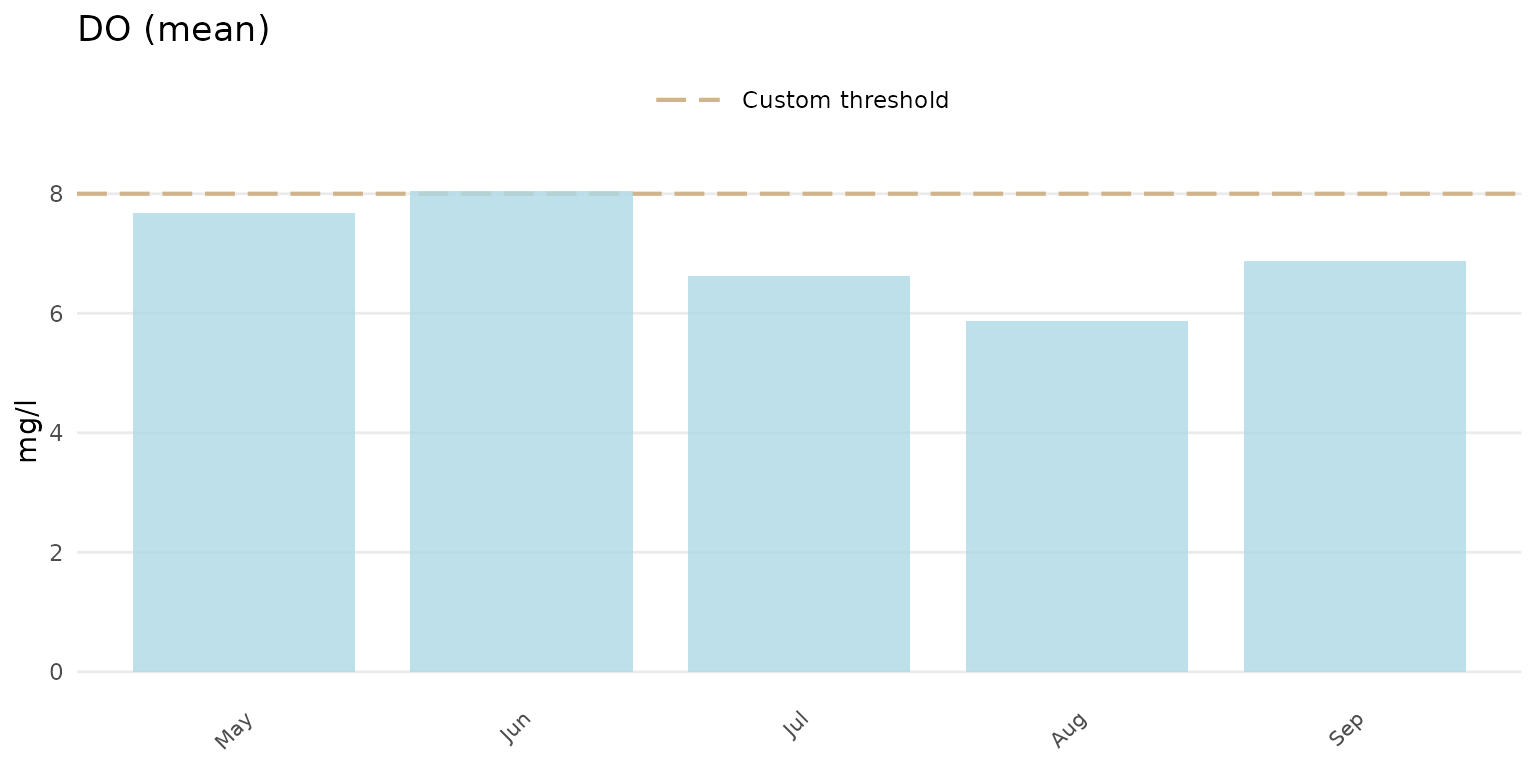The y-axis scaling as arithmetic (linear) or logarithmic can be set with the yscl argument. If yscl = "auto" (default), the scaling is determined automatically from the data quality objective file for accuracy, i.e., parameters with “log” in any of the columns are plotted on log10-scale, otherwise arithmetic. Setting yscl = "linear" or yscl = "log" will set the axis as linear or log10-scale, respectively, regardless of the information in the data quality objective file for accuracy. Below, the axis for E. Coli is plotted on the log-10 scale automatically. The y-axis scaling does not need to specified explicitly in the function call because the default setting is yscl = "auto".

anlzMWRseason(res = resdat, param = "E.coli", acc = accdat, thresh = "fresh", group = "month", type = "bar")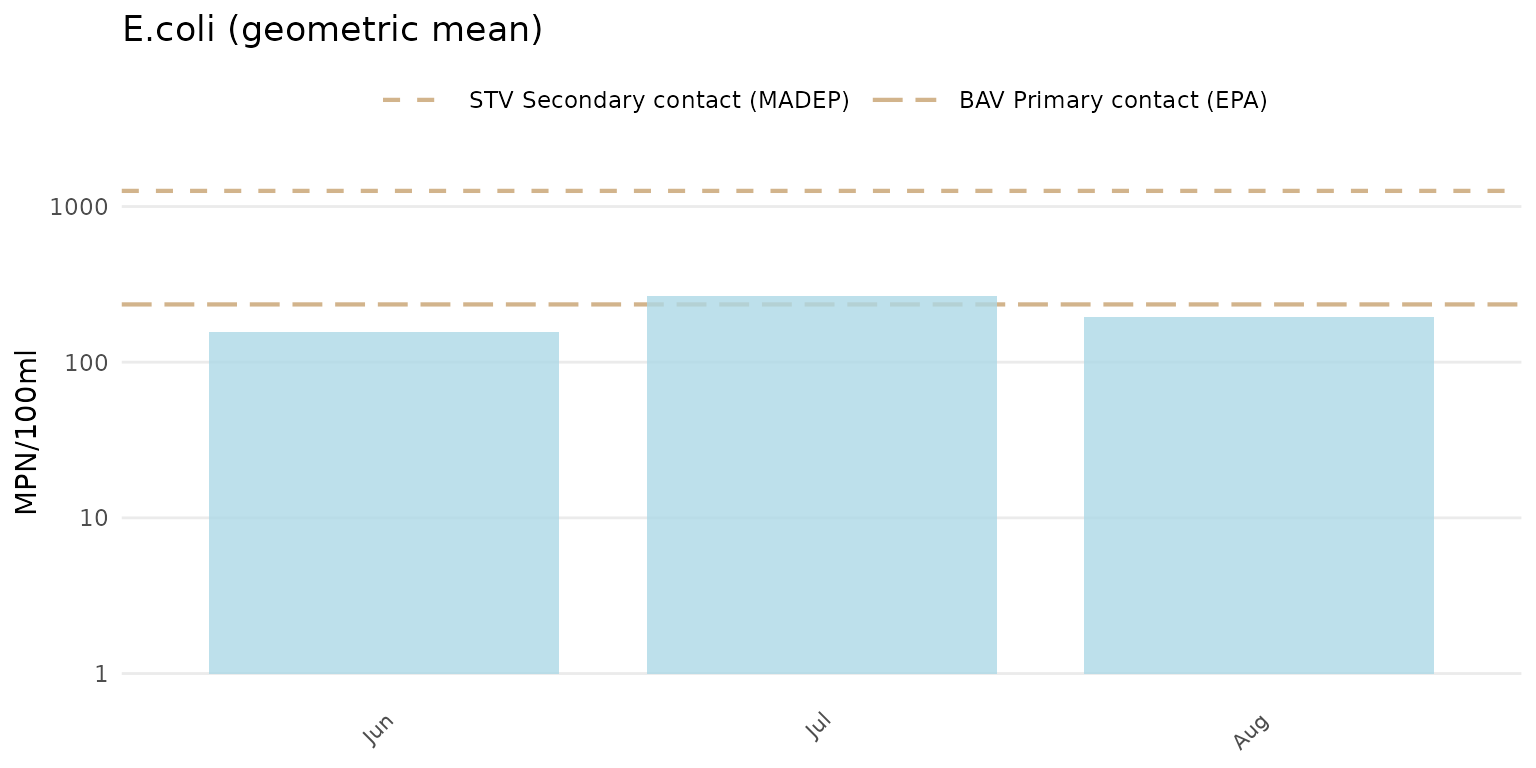To force the y-axis as linear for E. Coli, yscl = "linear" must be used. Note that the default linear scaling for dissolved oxygen above is determined automatically. The means and confidence intervals will also vary between arithmetic and log-scaling if type = "bar".

anlzMWRseason(res = resdat, param = "E.coli", acc = accdat, thresh = "fresh", group = "month", type = "bar", yscl = "linear")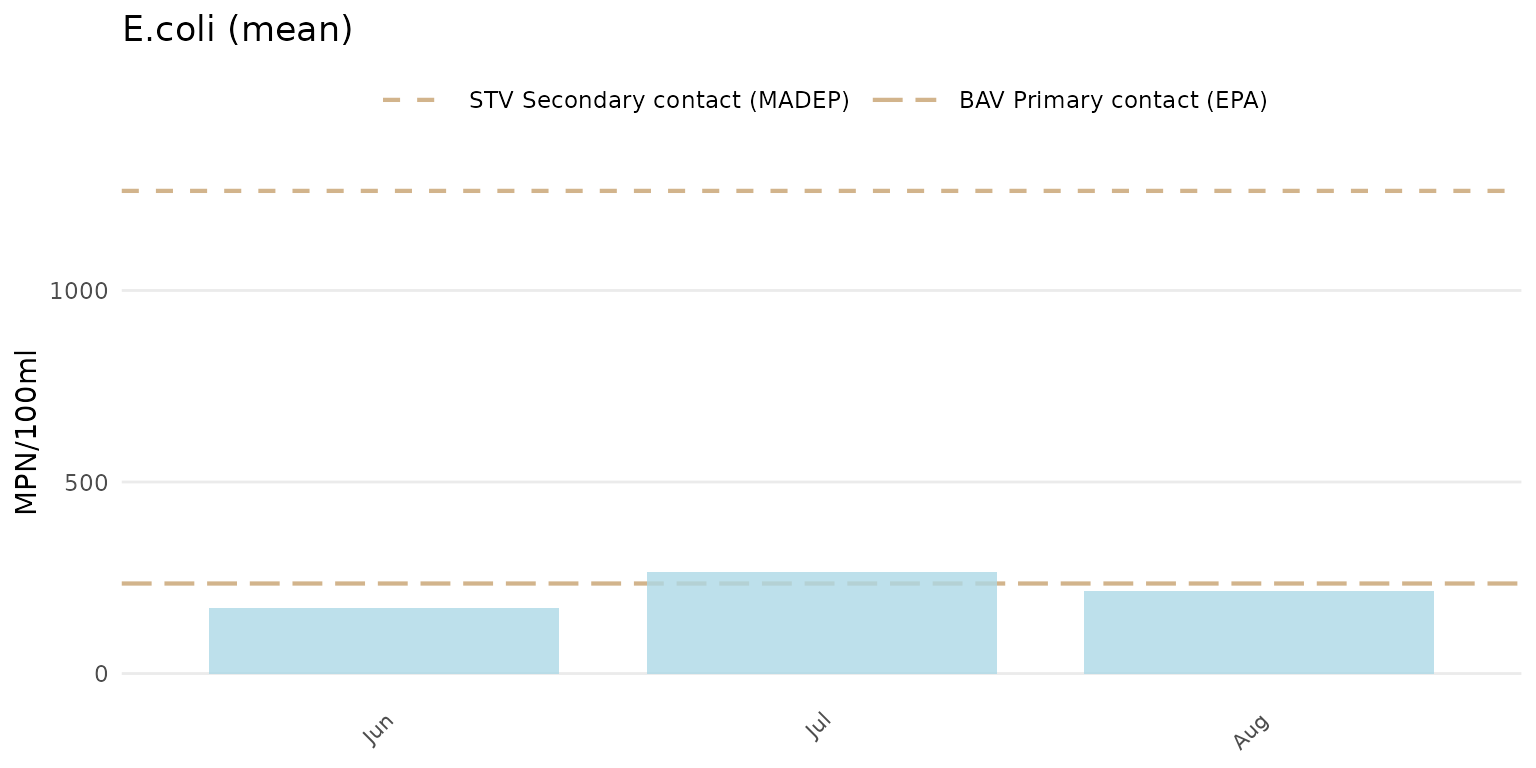If type is "bar" or "jitterbar", the data will be summarized using the mean or geometric mean as appropriate for the parameter based on information in the data quality objective file for accuracy. By default, the summary is based on the yscl argument. The default can be changed by passing a value to the sumfun argument, where appropriate values include "auto" (automatically as mean or geometric mean using information in the data quality objective file for accuracy), "mean", "geomean", "median", "min", or "max". Confidence intervals will be included if confint = TRUE and the summary is automatic or the mean or geometric mean is used to override the automatic summary. Below, the median summary is shown for E. coli.

anlzMWRseason(res = resdat, param = "E.coli", acc = accdat, thresh = "fresh", group = "month", type = "bar", sumfun = "median")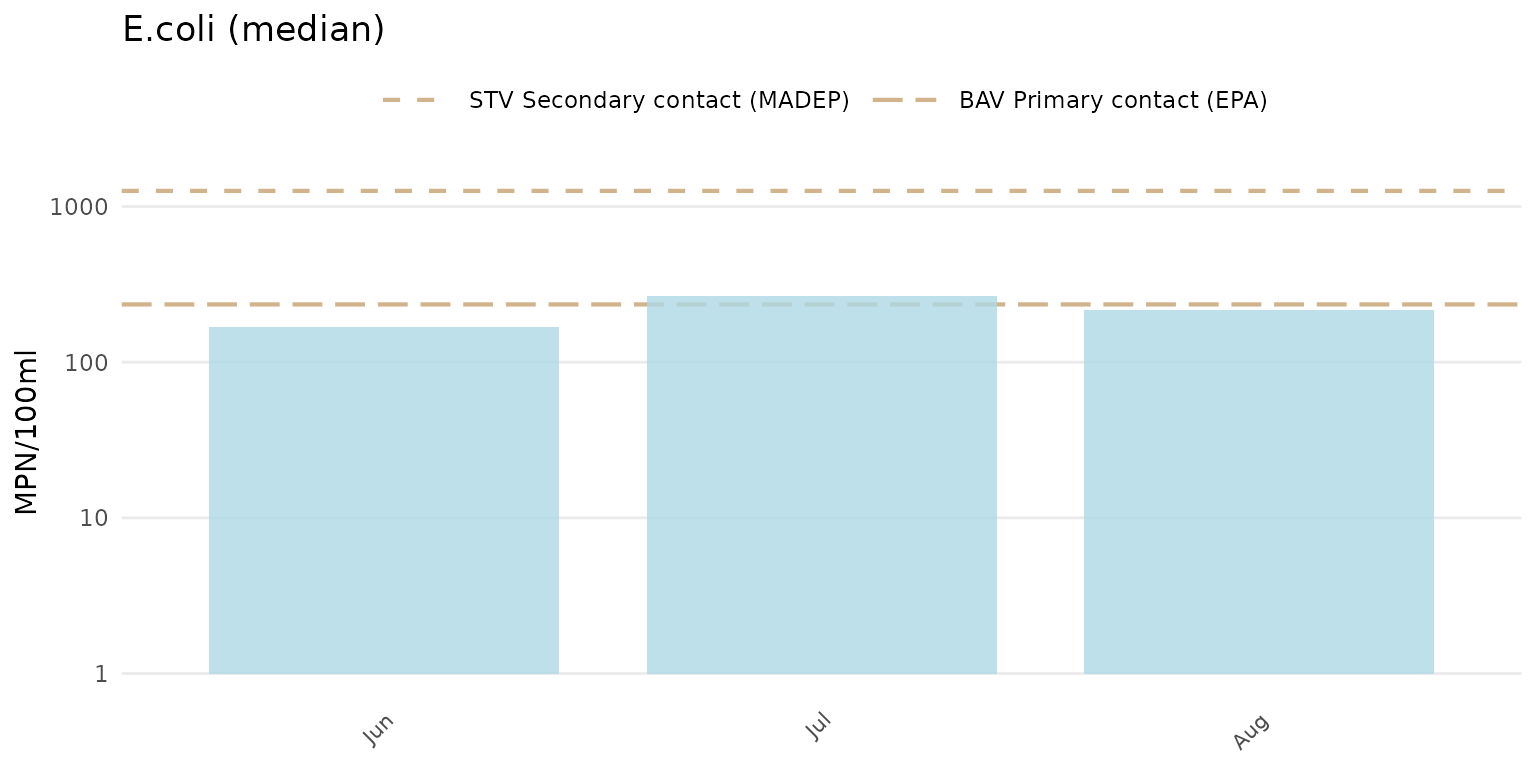Results can also be filtered by dates using the dtrng argument. The date format must be YYYY-MM-DD and include two entries.

anlzMWRseason(res = resdat, param = "E.coli", acc = accdat, thresh = "fresh", group = "month", type = "bar", dtrng = c("2022-05-01", "2022-07-31"))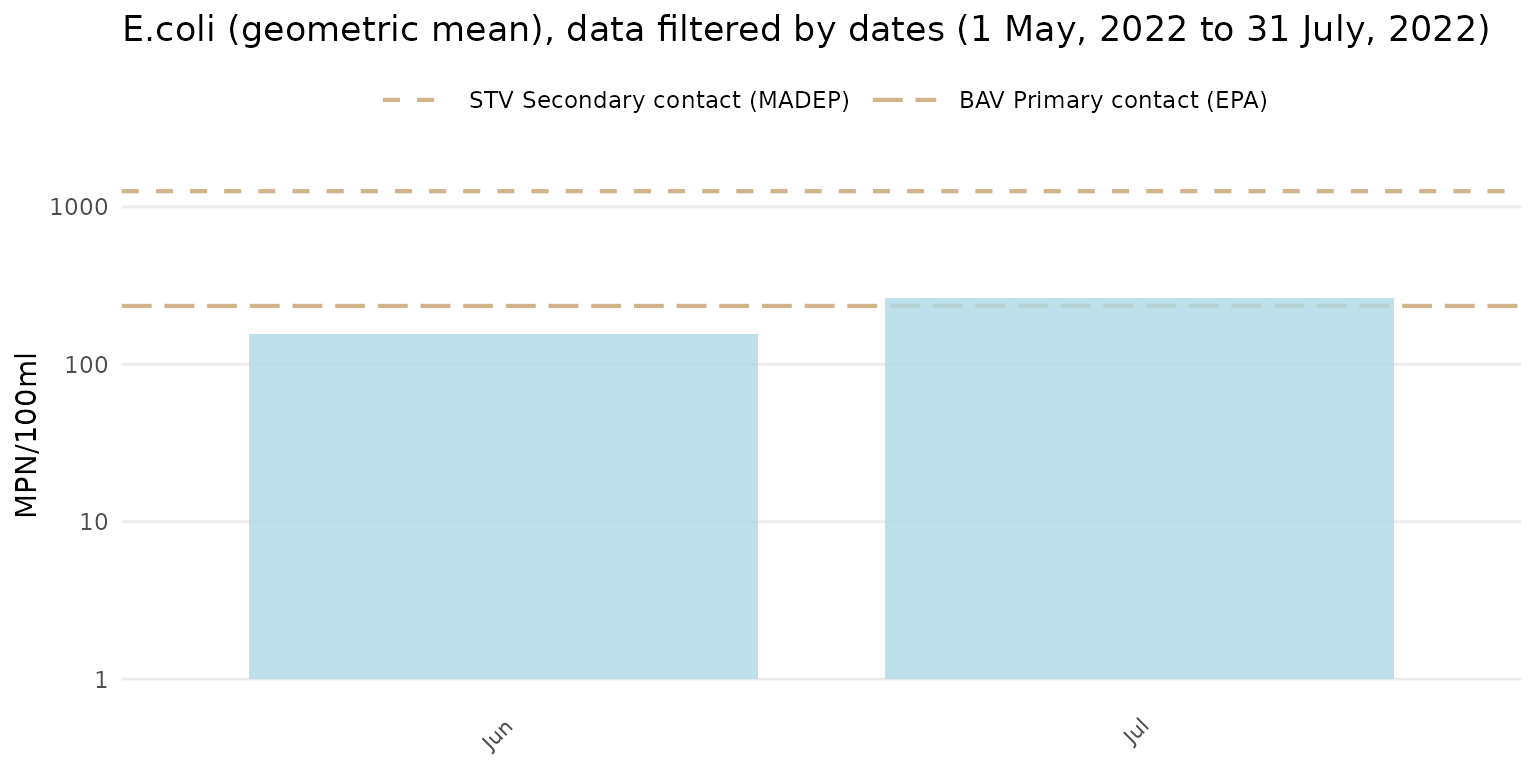Finally, the results can be filtered by sites, result attributes, and location groups using the site, resultatt, and locgroup arguments. These options will filter the results data based on values in the Monitoring Location ID and Result Attribute columns in the results file and by the Location Group column in the site metadata file, which must be provided to the sit argument if locgroup is used.

anlzMWRseason(res = resdat, param = "DO", acc = accdat, sit = sitdat, thresh = "fresh", group = "month", site = "ABT-077", resultatt = "DRY", locgroup = "Assabet")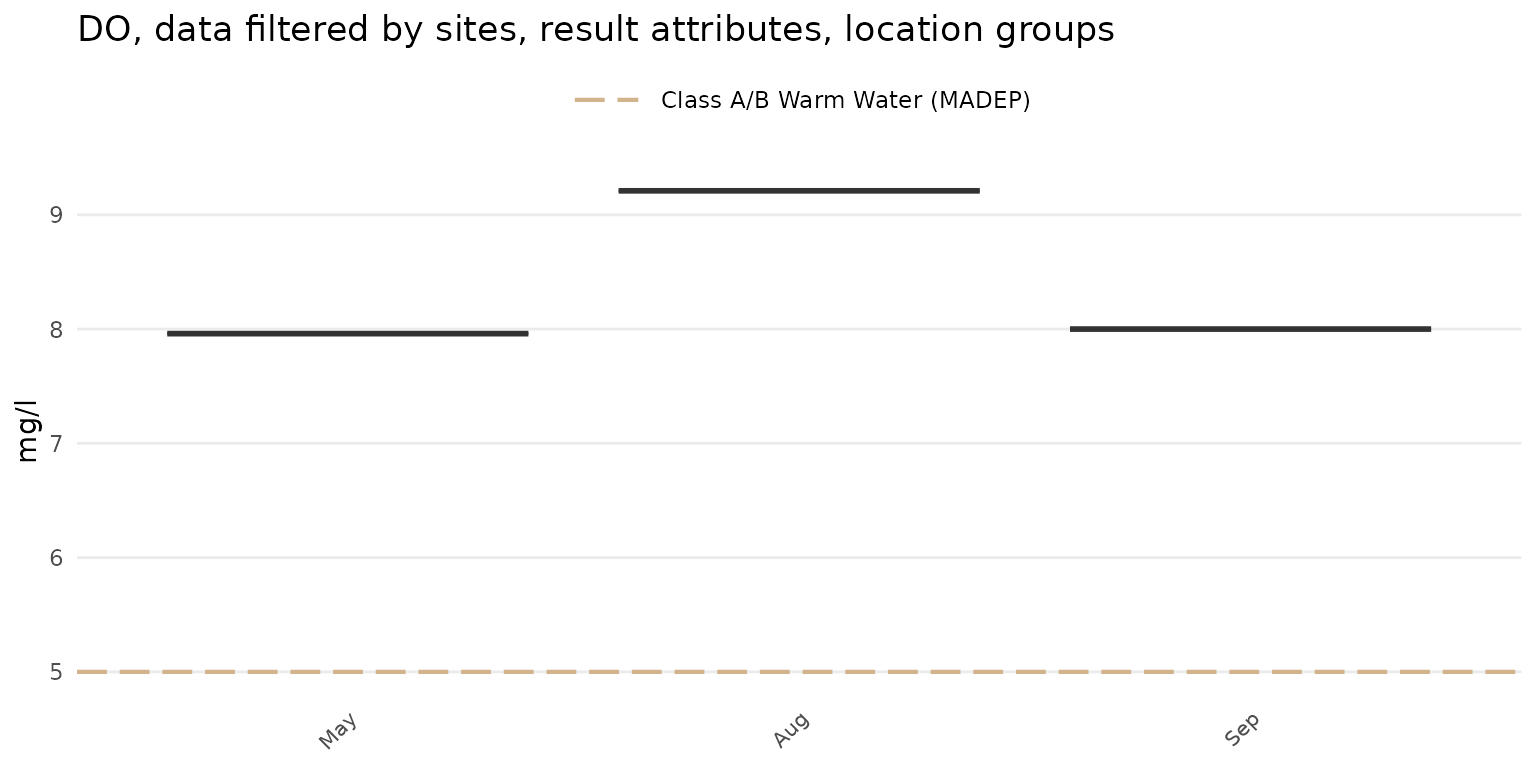Trends by date for selected parameters can be evaluated using the anlzMWRdate() function. The plot shows results continuously over time as line plots, with the lines separated by site, combined across sites, or combined across location groups. For location groups, the site metadata file must be supplied. Many of the options described for the anlzMWRseason() function also apply and will not be repeated here. As before, the required data are a results file and data quality objective file for accuracy. These can be passed to the function with the separate arguments or as a named list using the fset argument.

The default plot for anlzMWRdate() will show separate lines for each site, y-axis scaling determined automatically from the data quality objectives file, and site labels at the end of each line. All sites in the results file will be plotted, which can be difficult to visualize. Selecting specific sites with the site argument is recommended.

anlzMWRdate(res = resdat, param = "DO", acc = accdat, thresh = "fresh", group = "site", site = c("ABT-026", "ABT-077"))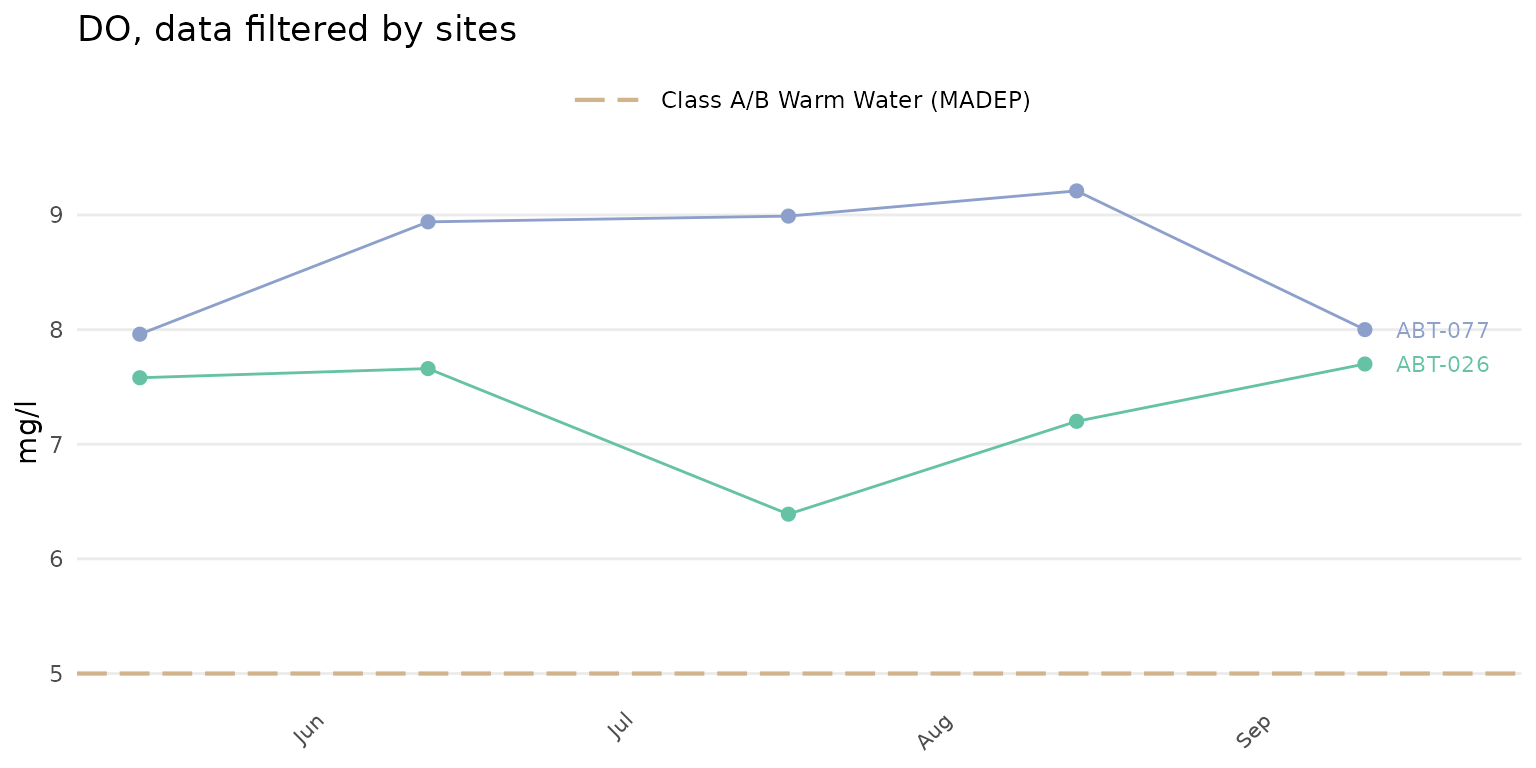A color legend for the sites can be included by setting colleg = TRUE.

anlzMWRdate(res = resdat, param = "DO", acc = accdat, thresh = "fresh", group = "site", site = c("ABT-026", "ABT-077"),
colleg = T)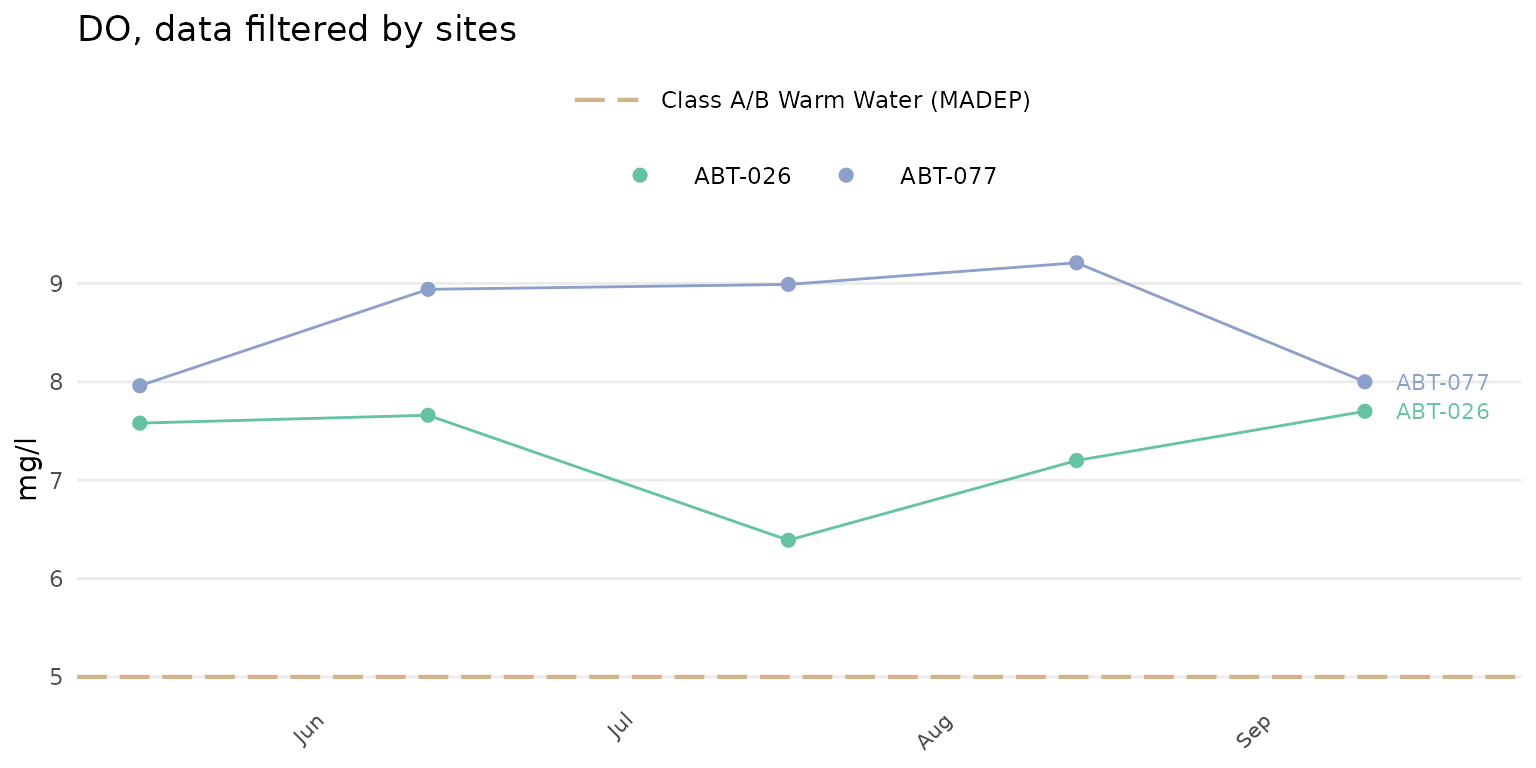The color palette for the site points and lines can also be changed. Any palette from RColorBrewer can be used. These could include any of the qualitative color palettes, e.g., "Set1", "Set2", etc. The continuous and diverging palettes will also work, but may return color scales for points and lines that are difficult to distinguish. The palcol argument does not apply if group = "all". In the following example, the qualitative "Set1" palette is used.

anlzMWRdate(res = resdat, param = "DO", acc = accdat, thresh = "fresh", group = "site", site = c("ABT-026", "ABT-077"), palcol = "Set1")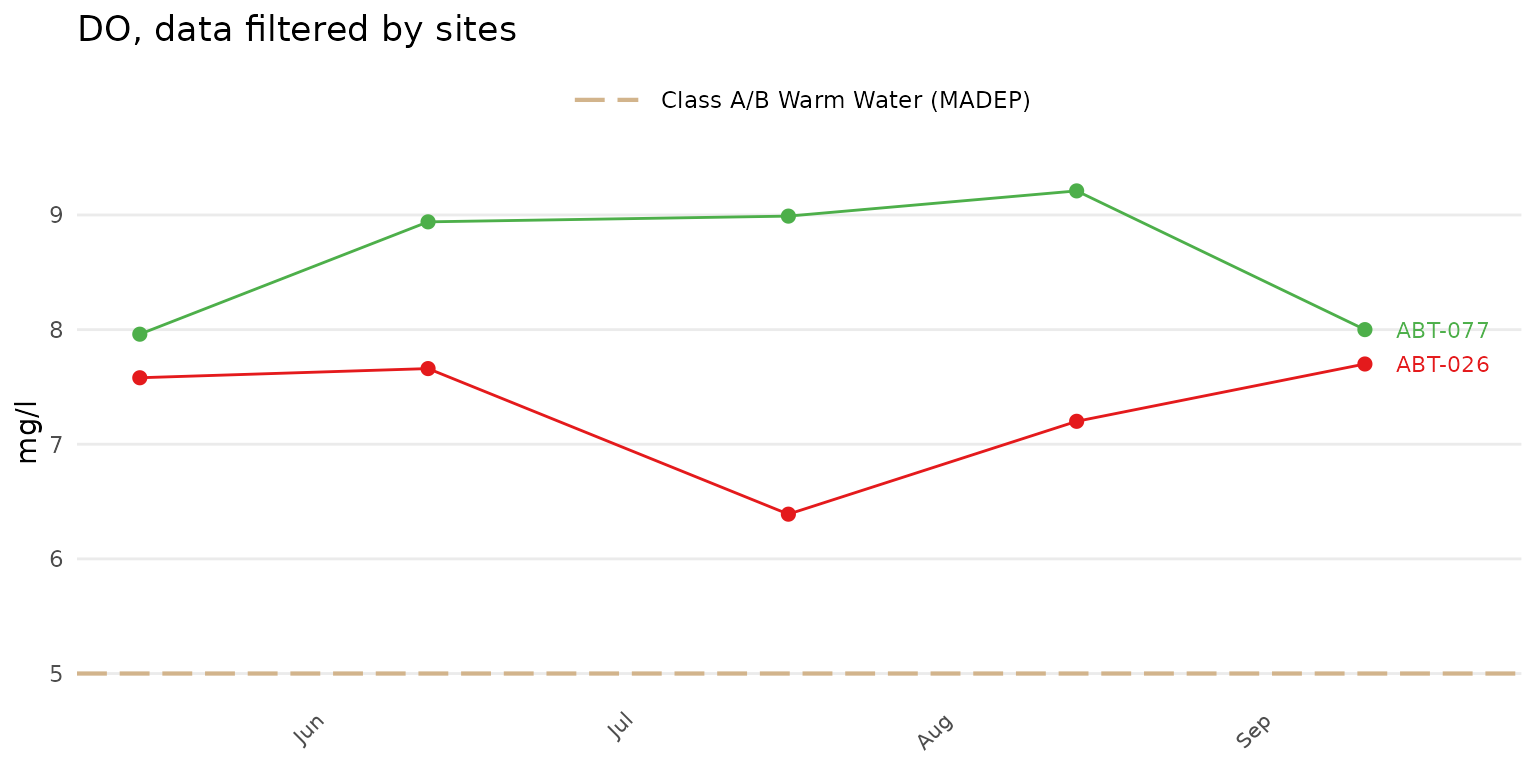The results averaged across the selected sites can be plotted using group = "all". The point and line color defaults to black.

anlzMWRdate(res = resdat, param = "DO", acc = accdat, thresh = "fresh", group = "all", site = c("ABT-026", "ABT-077"))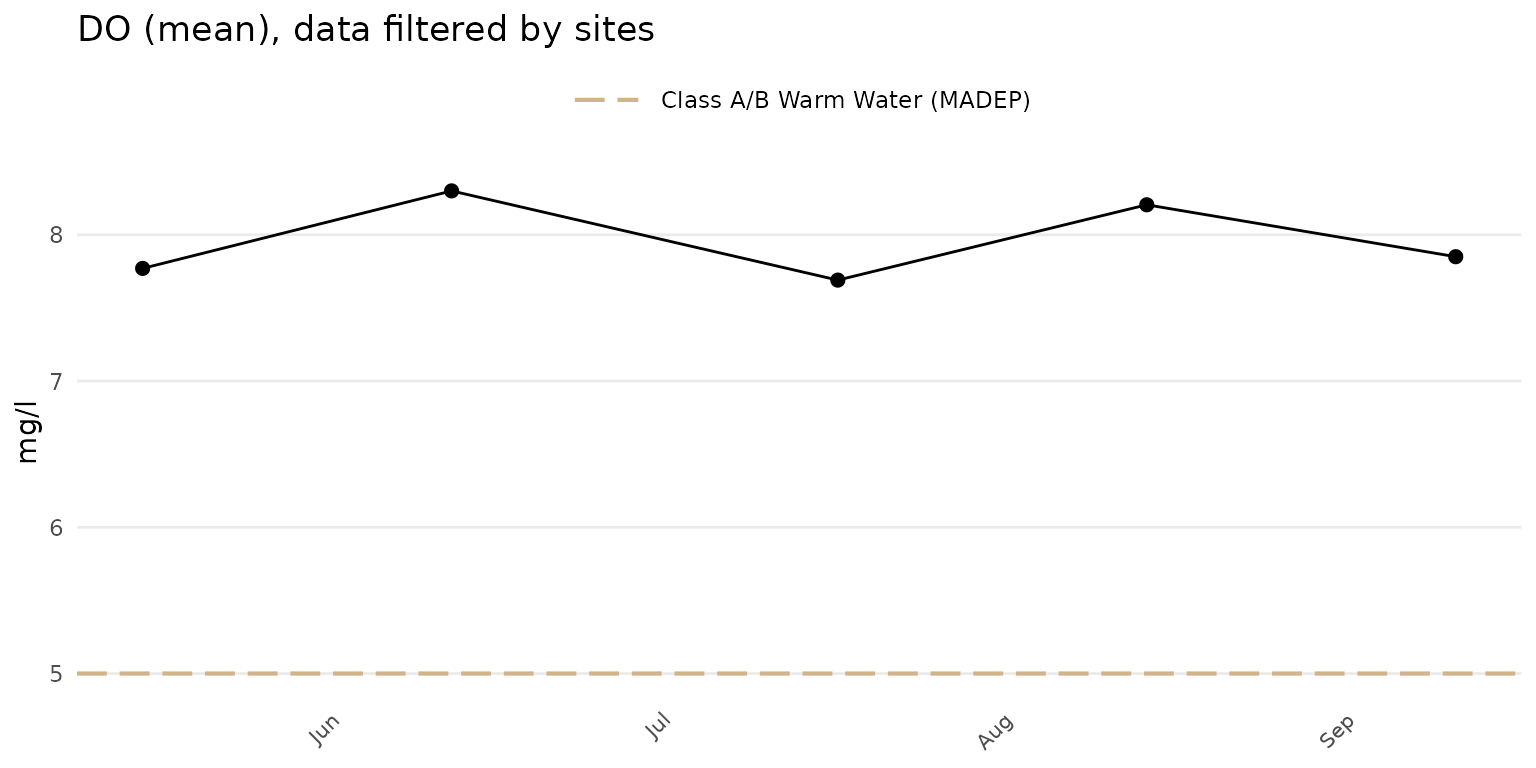The results averaged across all sites in the results file can be plotted by omitting the site argument.

anlzMWRdate(res = resdat, param = "DO", acc = accdat, thresh = "fresh", group = "all")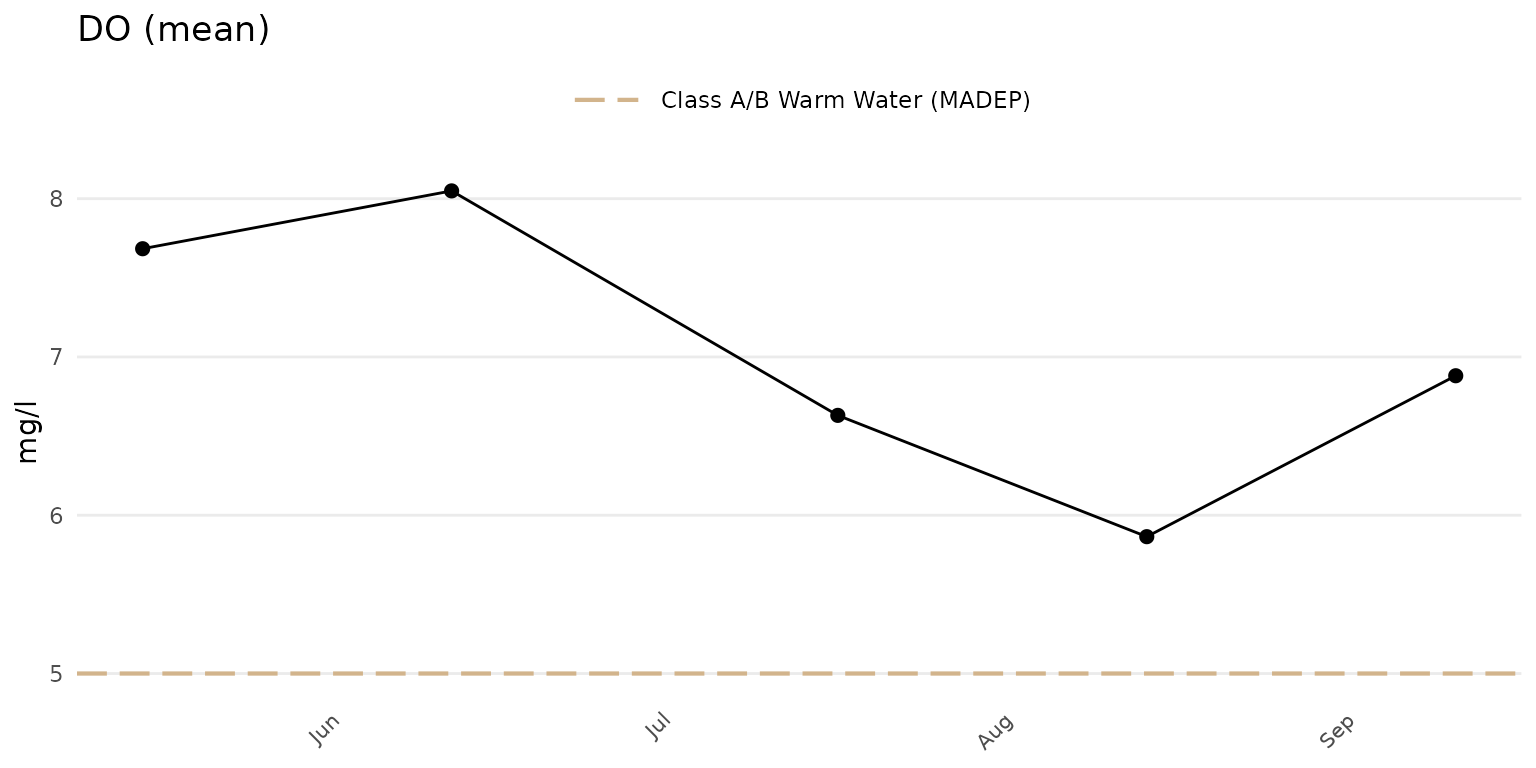Confidence intervals at 95% for the points can be shown when group = "all" or "locgroup" by setting confint = TRUE.

anlzMWRdate(res = resdat, param = "DO", acc = accdat, thresh = "fresh", group = "all", confint = TRUE)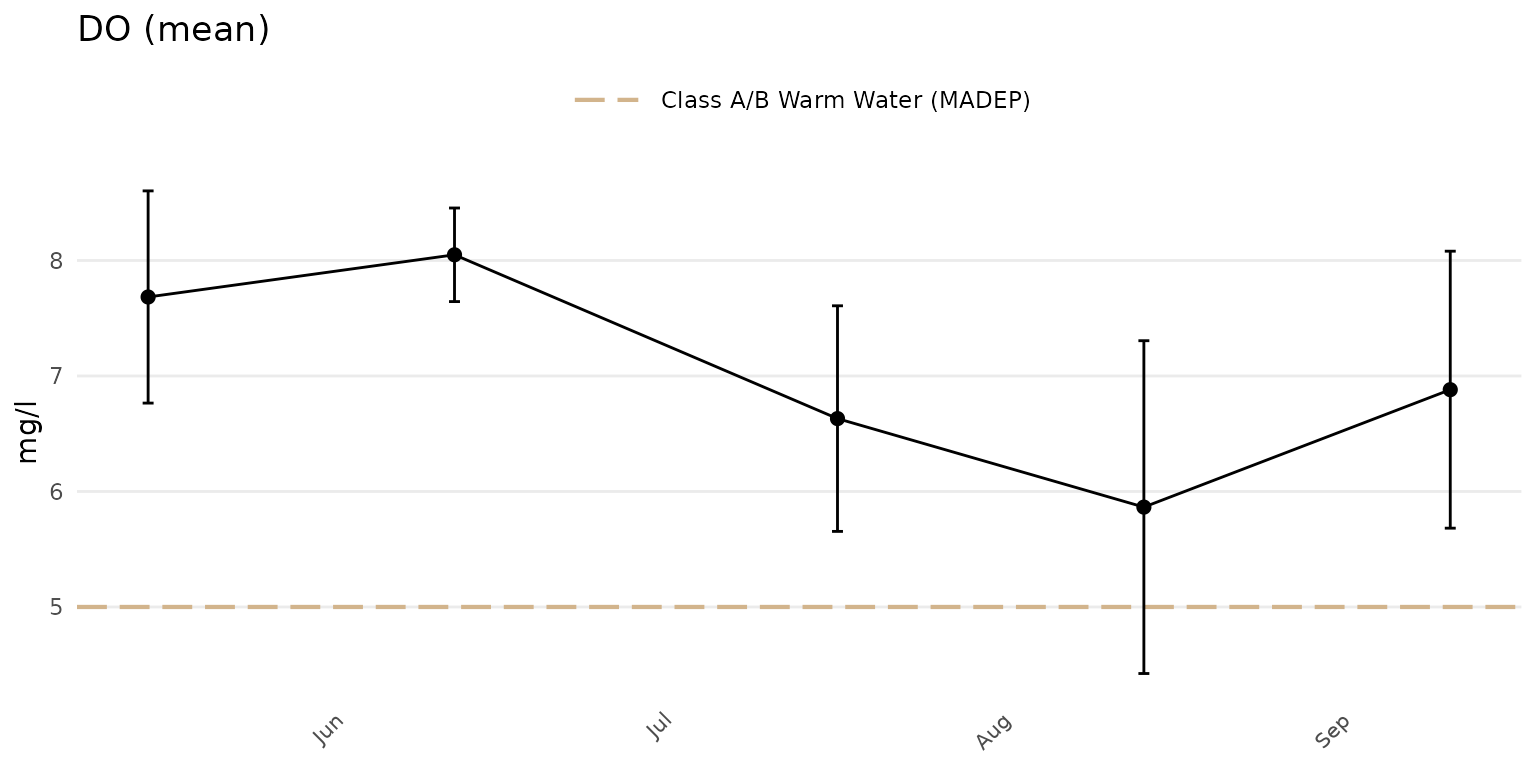Sites can be summarized across location groups using group = "locgroup". This option requires the site metadata file passed to the sit argument and appropriate values for the location groups passed to the locgroup argument that match values in the Location Group column of the site metadata file.

anlzMWRdate(res = resdat, param = 'DO', acc = accdat, sit = sitdat, group = 'locgroup',
thresh = 'fresh', locgroup = 'Assabet')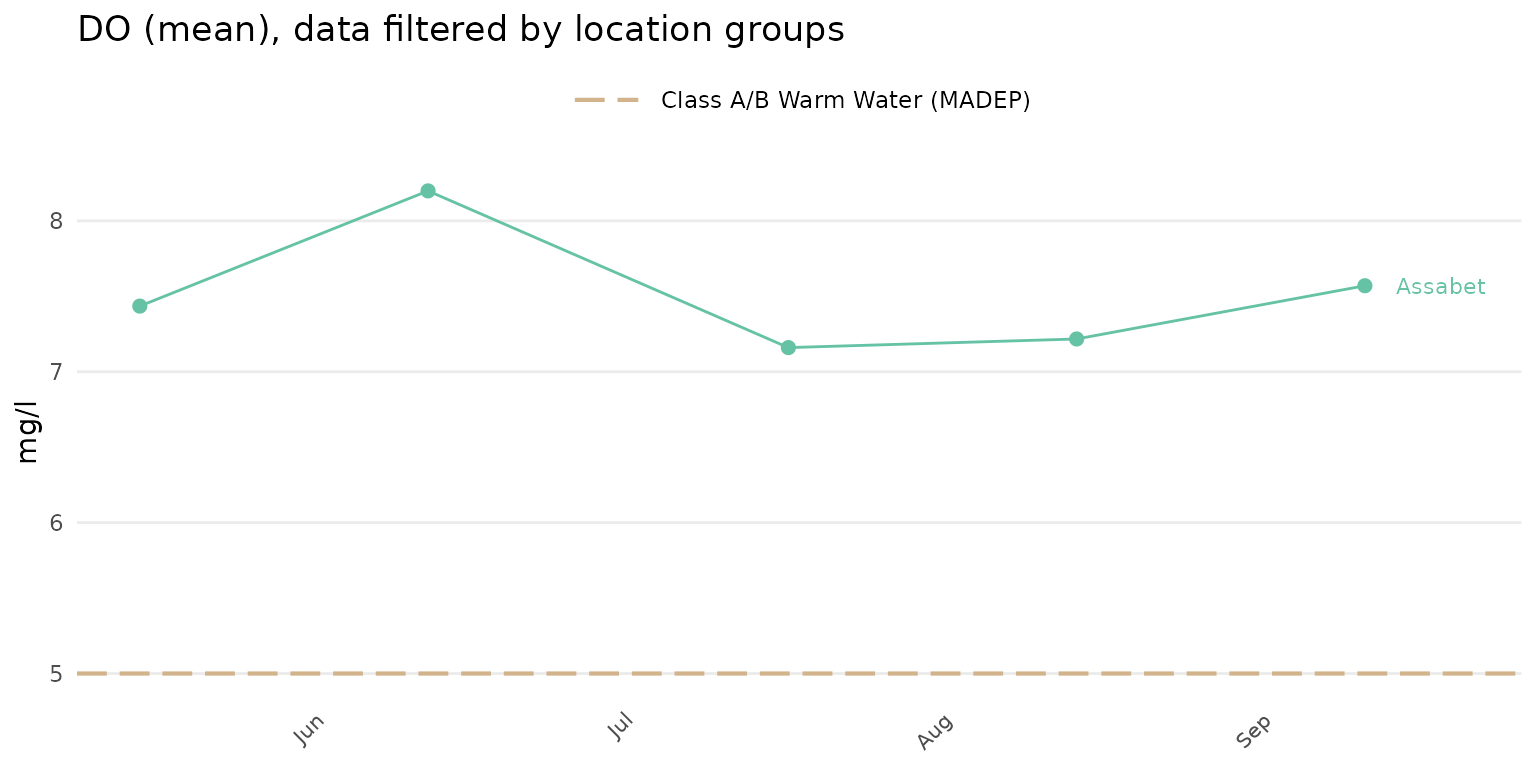All location groups can be used if the locgroup argument is omitted.

anlzMWRdate(res = resdat, param = 'DO', acc = accdat, sit = sitdat, group = 'locgroup',
thresh = 'fresh')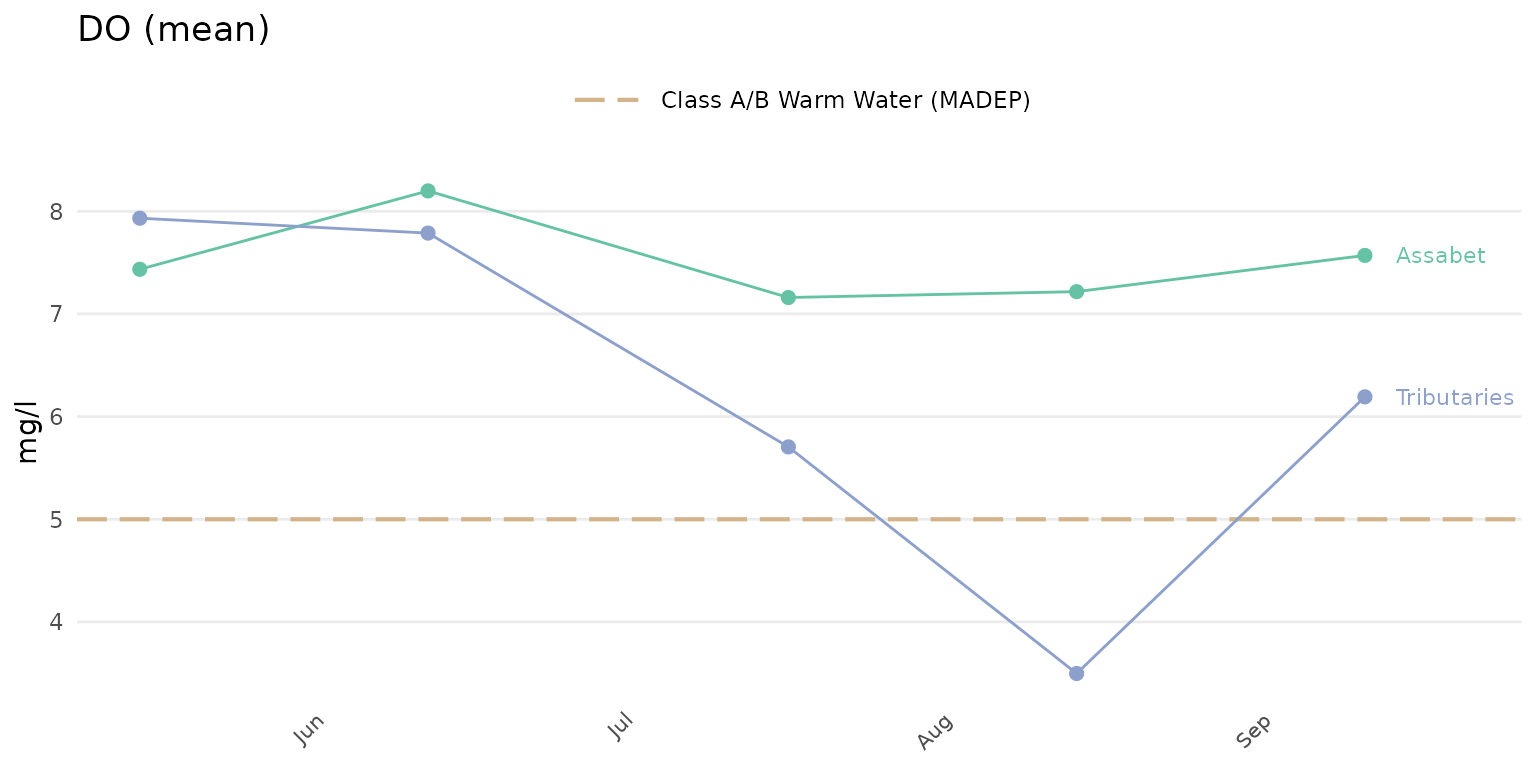Alternative summaries can also be used if group = "all" or "locgroup" by using an appropriate value for the sumfun argument. By default, the sumfun argument is based on the value passed to yscl, i.e., automatic summaries (yscl = "auto") as mean or geometric mean based on information in the data quality objective file for accuracy or using mean or geometric regardless of the accuracy file (yscl = "linear" or yscl = "log"). Using any appropriate value for the sumfun argument will override any value used for the yscl argument. Options for sumfun include "auto" (automatically as mean or geometric mean using information in the data quality objective file for accuracy), "mean", "geomean", "median", "min", or "max". Below, the median is used to summarize results by location group.

anlzMWRdate(res = resdat, param = 'DO', acc = accdat, sit = sitdat, group = 'locgroup',
thresh = 'fresh', sumfun = 'median')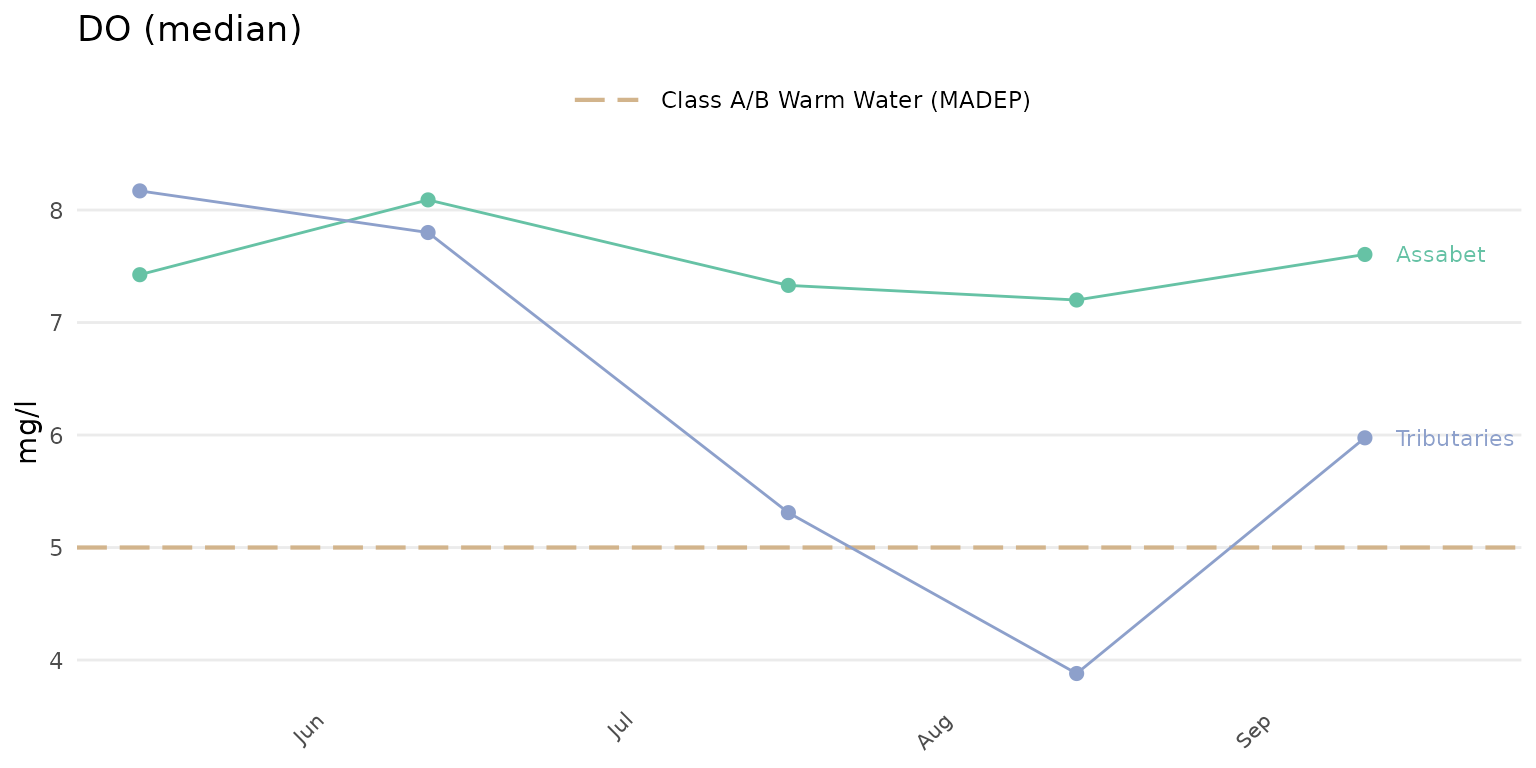## Analyze data by site

Result data by site for selected parameters can be evaluated using the anlzMWRsite() function. This function summarizes results for a single parameter using boxplots or barplots separately for each site on the x-axis. Boxplots or barplots can also include jittered points of the observations on top or only the jittered points can be shown. Many of the options described for the anlzMWRseason() function also apply and will not be repeated here. As before, the required data are a results file and data quality objective file for accuracy. These can be passed to the function with the separate arguments or as a named list using the fset argument.

The default plot for anlzMWRsite() will show boxplots of results for each site and y-axis scaling determined automatically from the data quality objectives file.

anlzMWRsite(res = resdat, param = "DO", acc = accdat, thresh = "fresh", type = "box")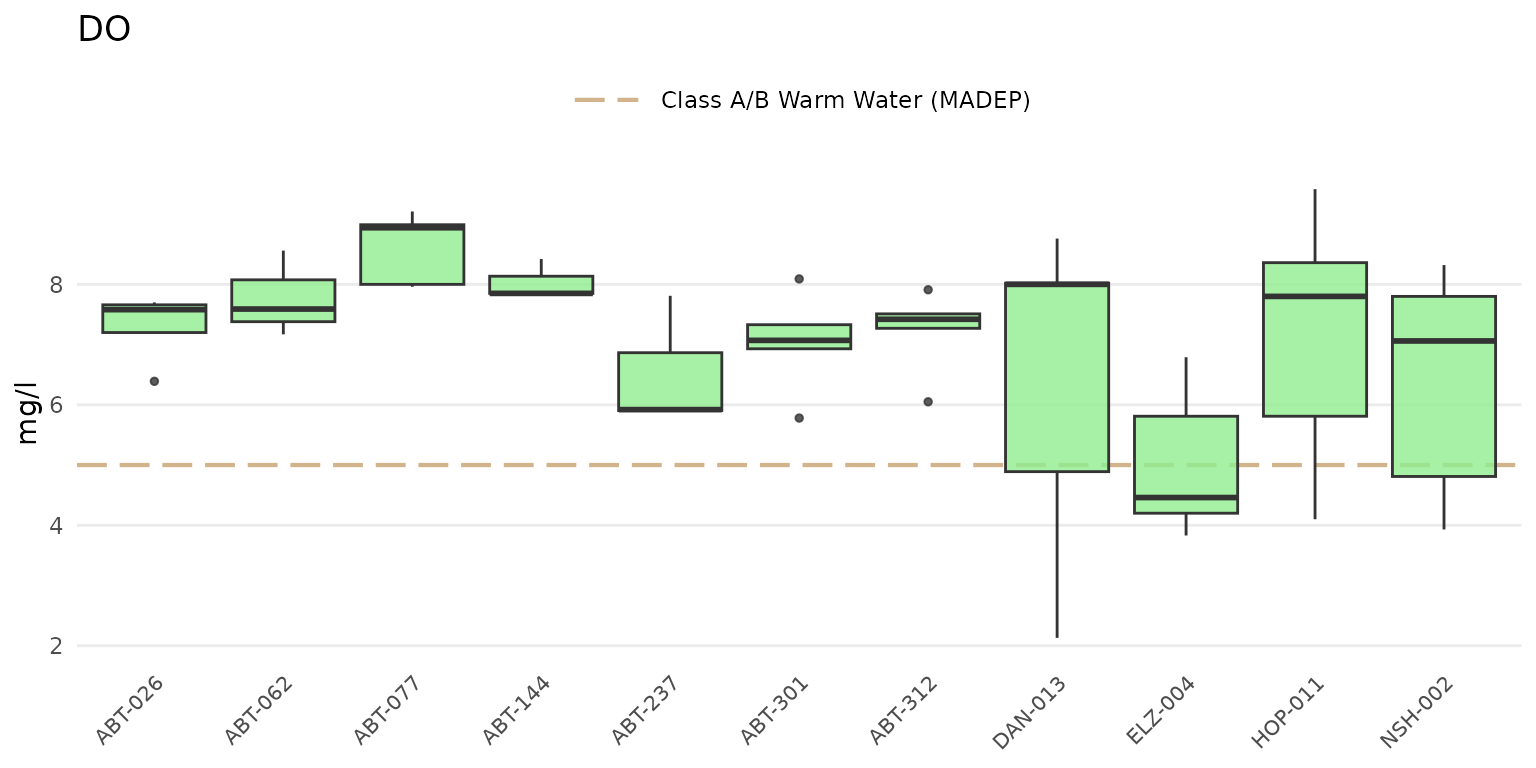Jittered points over the boxplots can be shown by setting type = "jitterbox".

anlzMWRsite(res = resdat, param = "DO", acc = accdat, thresh = "fresh", type = "jitterbox")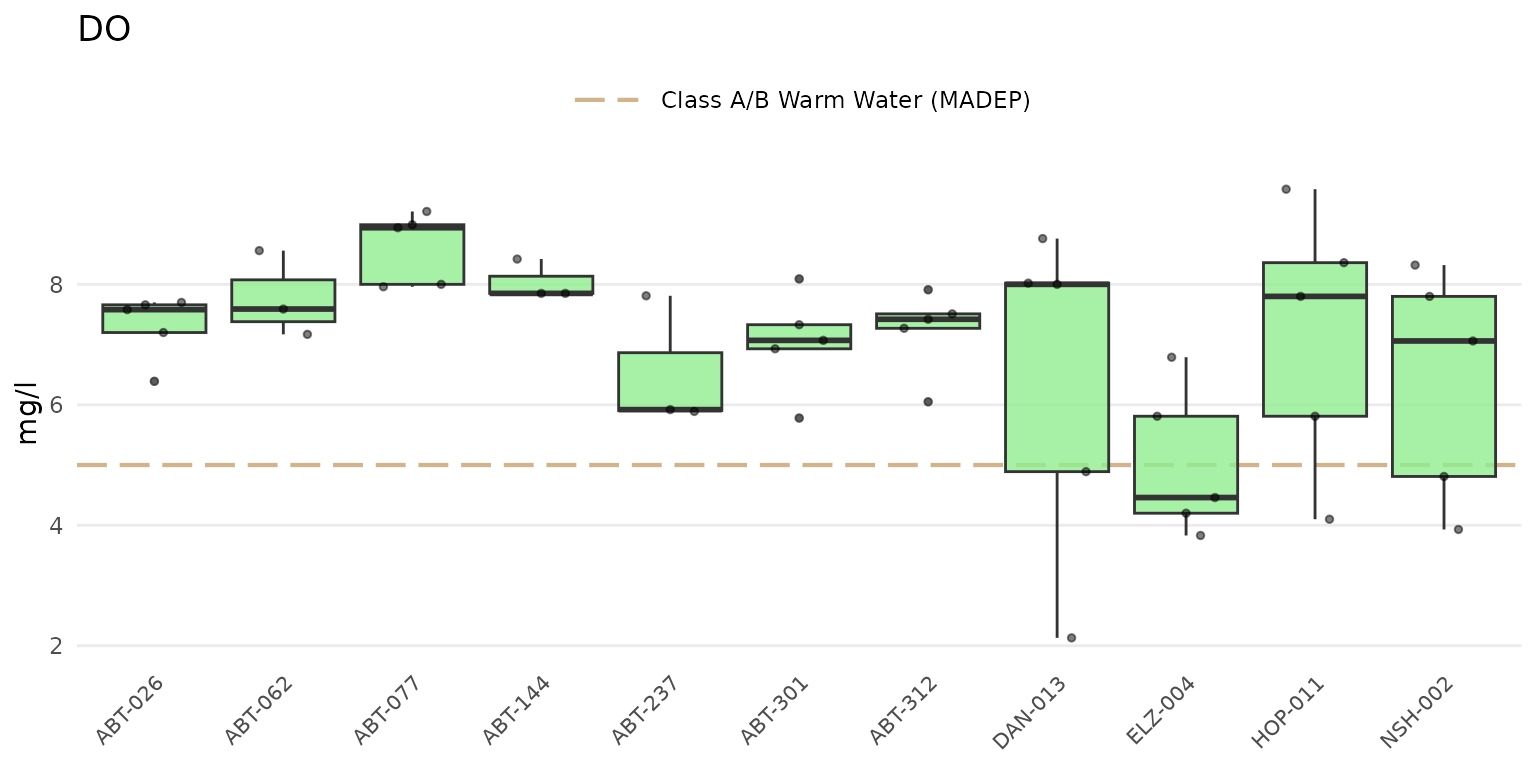Results as barplots can be shown using type = "bar".

anlzMWRsite(res = resdat, param = "DO", acc = accdat, thresh = "fresh", type = "bar")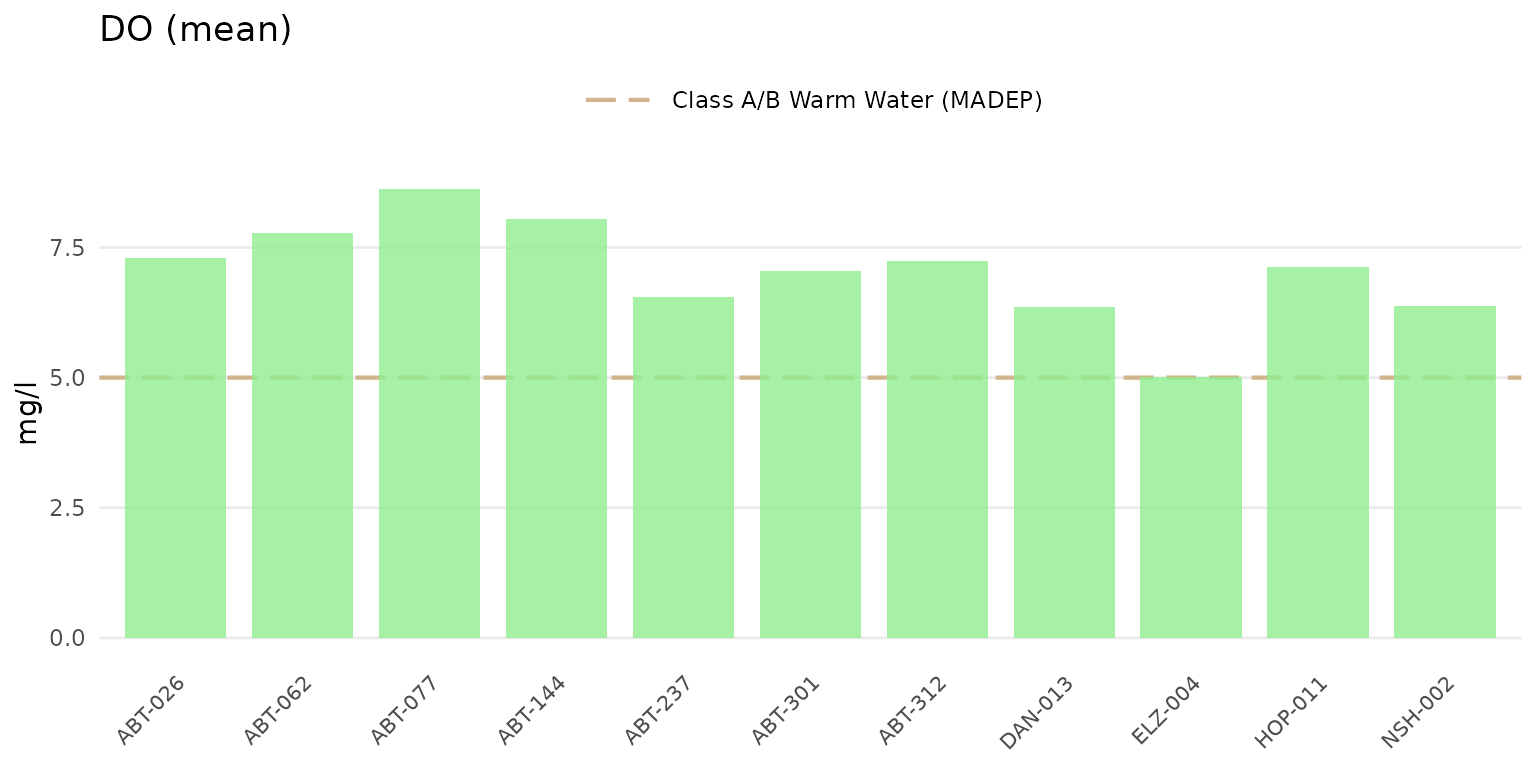Confidence intervals at 95% for the barplots can be shown by setting confint = TRUE.

anlzMWRsite(res = resdat, param = "DO", acc = accdat, thresh = "fresh", type = "bar", confint = TRUE)Jittered points over the barplots can be shown by setting type = "jitterbar".

anlzMWRsite(res = resdat, param = "DO", acc = accdat, thresh = "fresh", type = "jitterbar")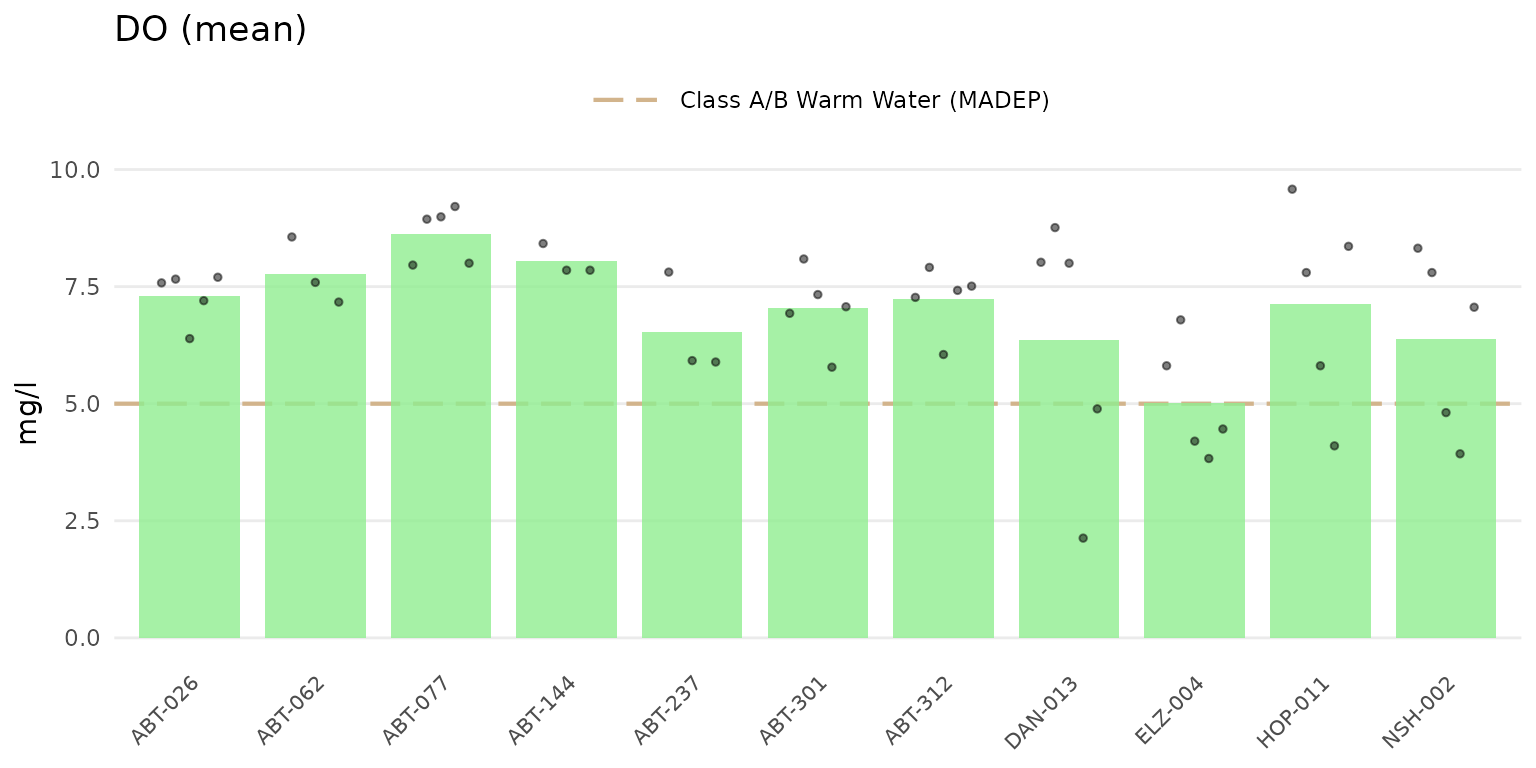Setting type = "jitter" will show only the jittered points.

anlzMWRsite(res = resdat, param = "DO", acc = accdat, thresh = "fresh", type = "jitter")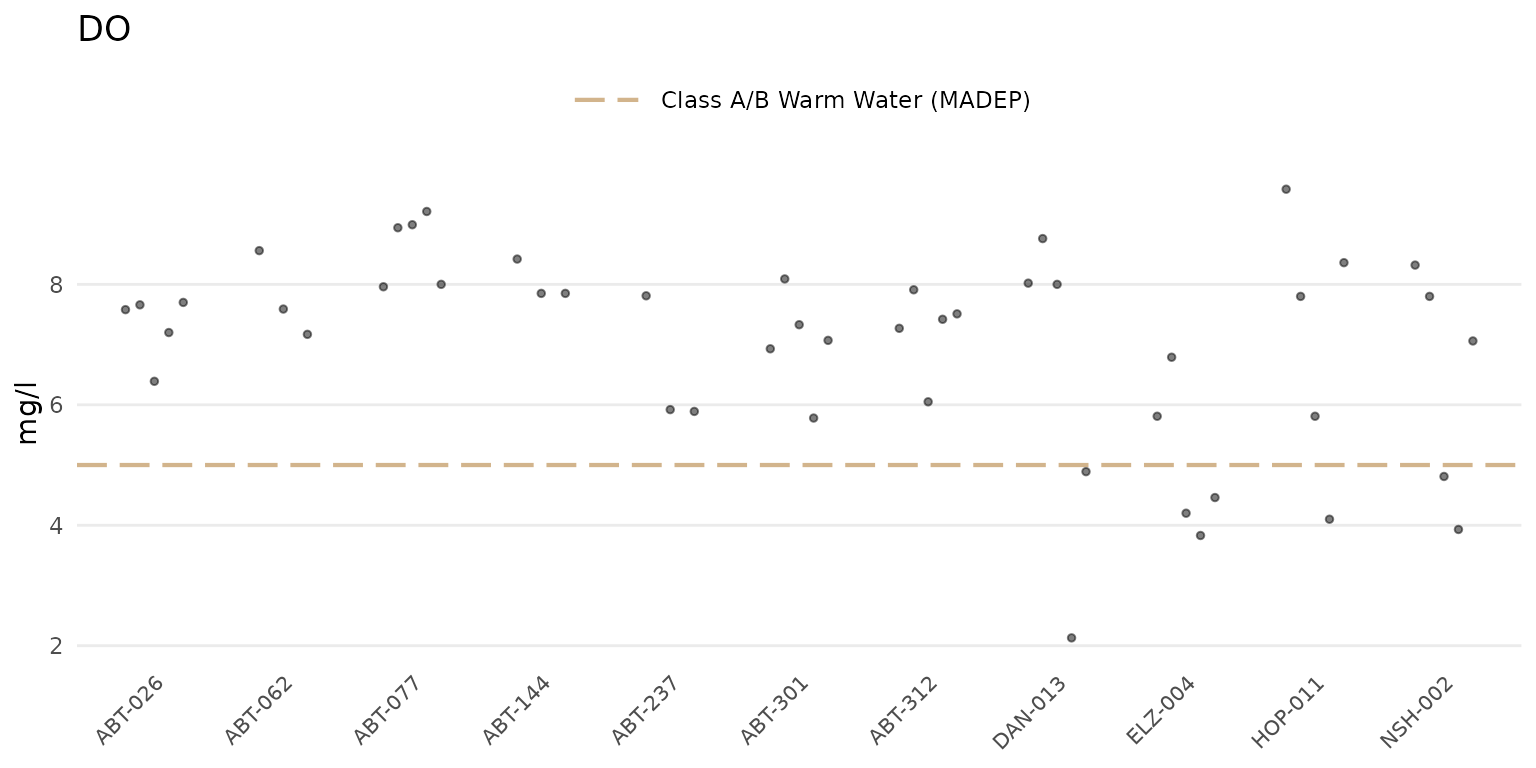If type is "bar" or "jitterbar", the data will be summarized using the mean or geometric mean as appropriate for the parameter based on information in the data quality objective file for accuracy. By default, the summary is based on the yscl argument. The default can be changed by passing a value to the sumfun argument, where appropriate values include "auto" (automatically as mean or geometric mean using information in the data quality objective file for accuracy), "mean", "geomean", "median", "min", or "max". Below, the median summary is shown.

anlzMWRsite(res = resdat, param = "DO", acc = accdat, thresh = "fresh", type = "jitterbar",
sumfun = 'median')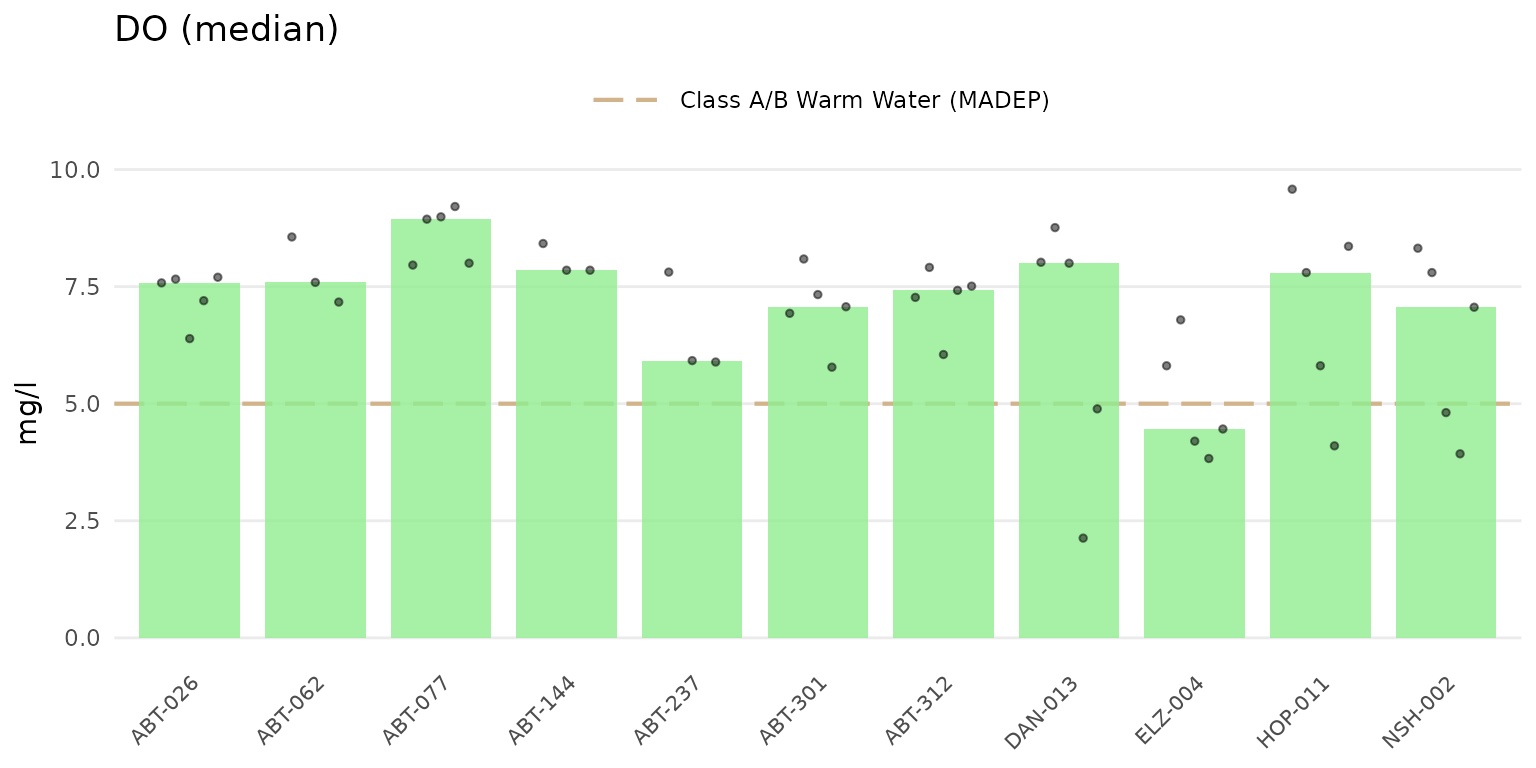Results can be grouped by entries in the Result Atribute column using byresultatt = TRUE. For example, sites with DO samples can be grouped by "DRY" or "WET" conditions. Filtering by sites first using the site argument is advised to reduce the amount of data that are plotted. The grouping can be filtered further by passing appropriate values in the Result Attribute column to the resultatt argument. Note that specifying result attributes with resultatt and setting byresultatt = FALSE will filter the plot data by the result attributes but will not plot the results separately.

anlzMWRsite(res = resdat, param = "DO", acc = accdat, thresh = "fresh", type = "box",
site = c("ABT-026", "ABT-062", "ABT-077", "ABT-144", "ABT-237", "ABT-301"),
resultatt = c('DRY', 'WET'), byresultatt = TRUE)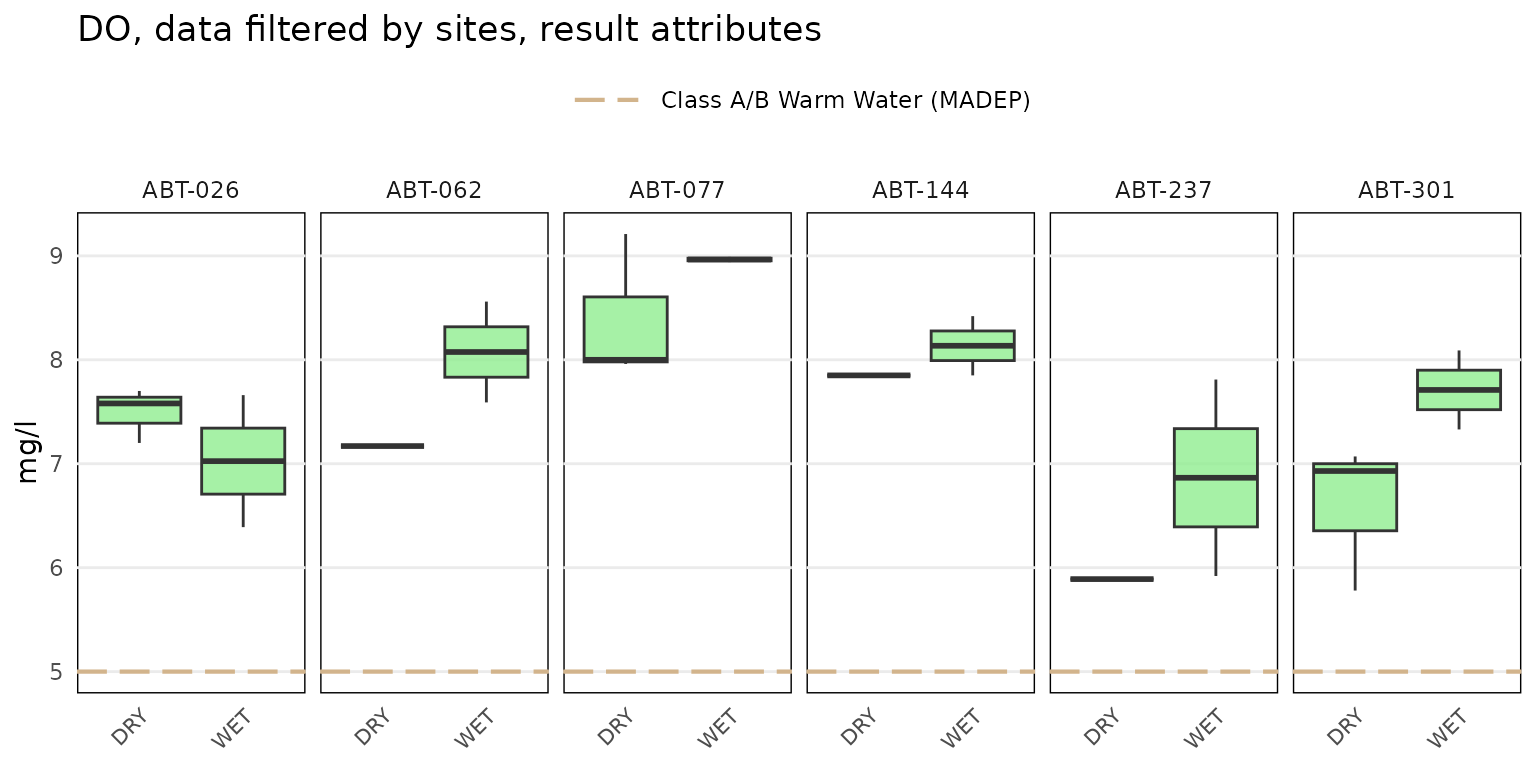## Analyze results with maps

The anlzMWRmap() function can be used to create a map of summarized results for a selected parameter at each monitoring site. By default, all dates for the parameter are summarized as the mean or geometric mean based on information in the data quality objective file for accuracy. Options to filter by site, date range, and result attribute are provided. Only sites with spatial information in the site metadata file are plotted and a warning is returned for those that do not have this information. The site labels are also plotted next to each point.

A map of dissolved oxygen averages across all dates can be created as follows. The required inputs are the results data, the data quality objective file for accuracy, and the site metadata file with the site locations. These can be passed to the function with the separate arguments or as a named list using the fset argument.

anlzMWRmap(res = resdat, param = "DO", acc = accdat, sit = sitdat, addwater = "medium")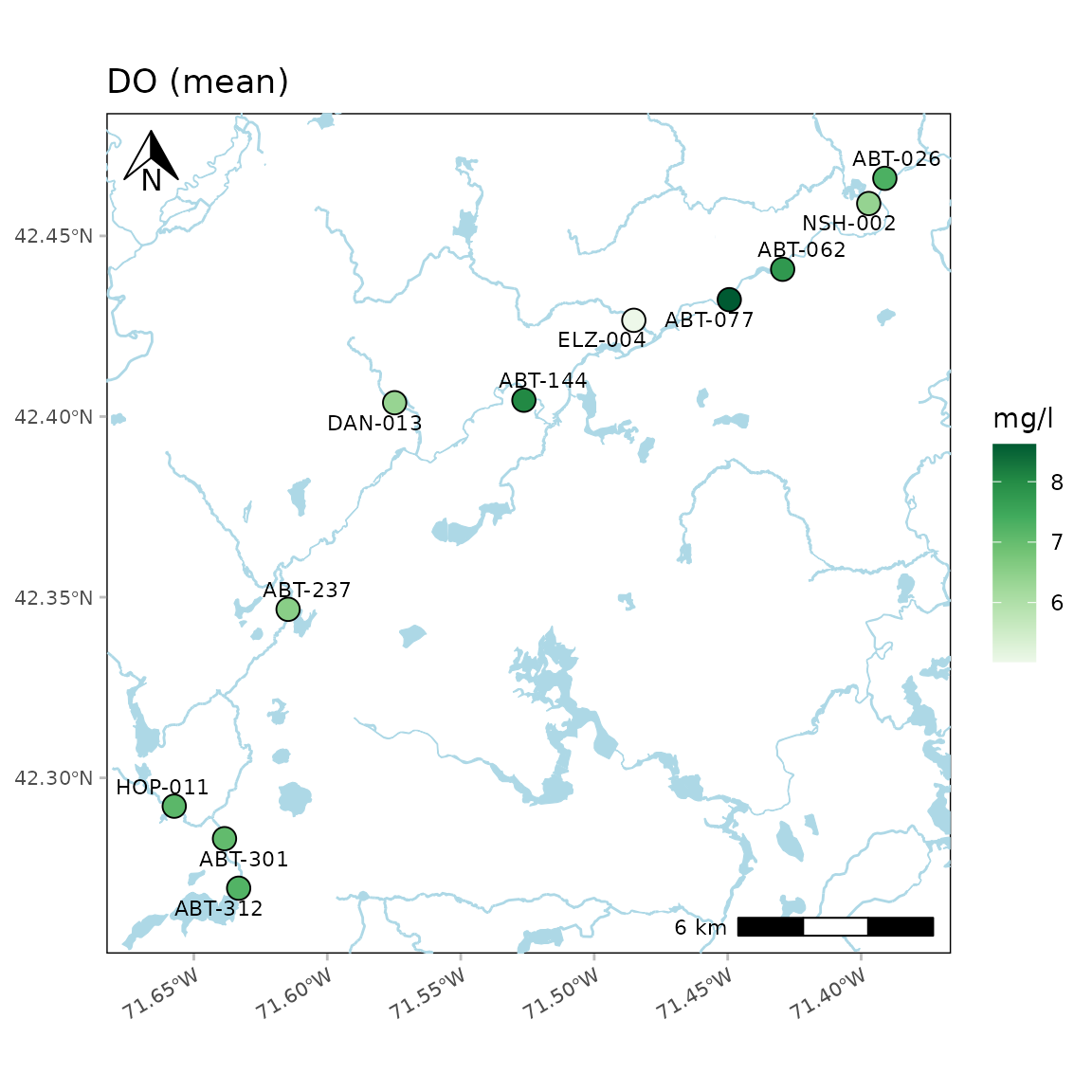The results shown on the map represent the parameter summary for each site across the sample dates. If sumfun = "auto" (default), the mean is used where the distribution is determined automatically from the data quality objective file for accuracy, i.e., parameters with “log” in any of the columns are summarized with the geometric mean, otherwise arithmetic. Any other valid summary function will be applied if passed to sumfun ("mean", "geomean", "median", "min", "max"), regardless of the information in the data quality objective file for accuracy. Below, the minimum dissolved oxygen is shown. The color palette is also reversed.

anlzMWRmap(res = resdat, param = "DO", acc = accdat, sit = sitdat, addwater = "medium",
sumfun = "min", palcolrev = TRUE)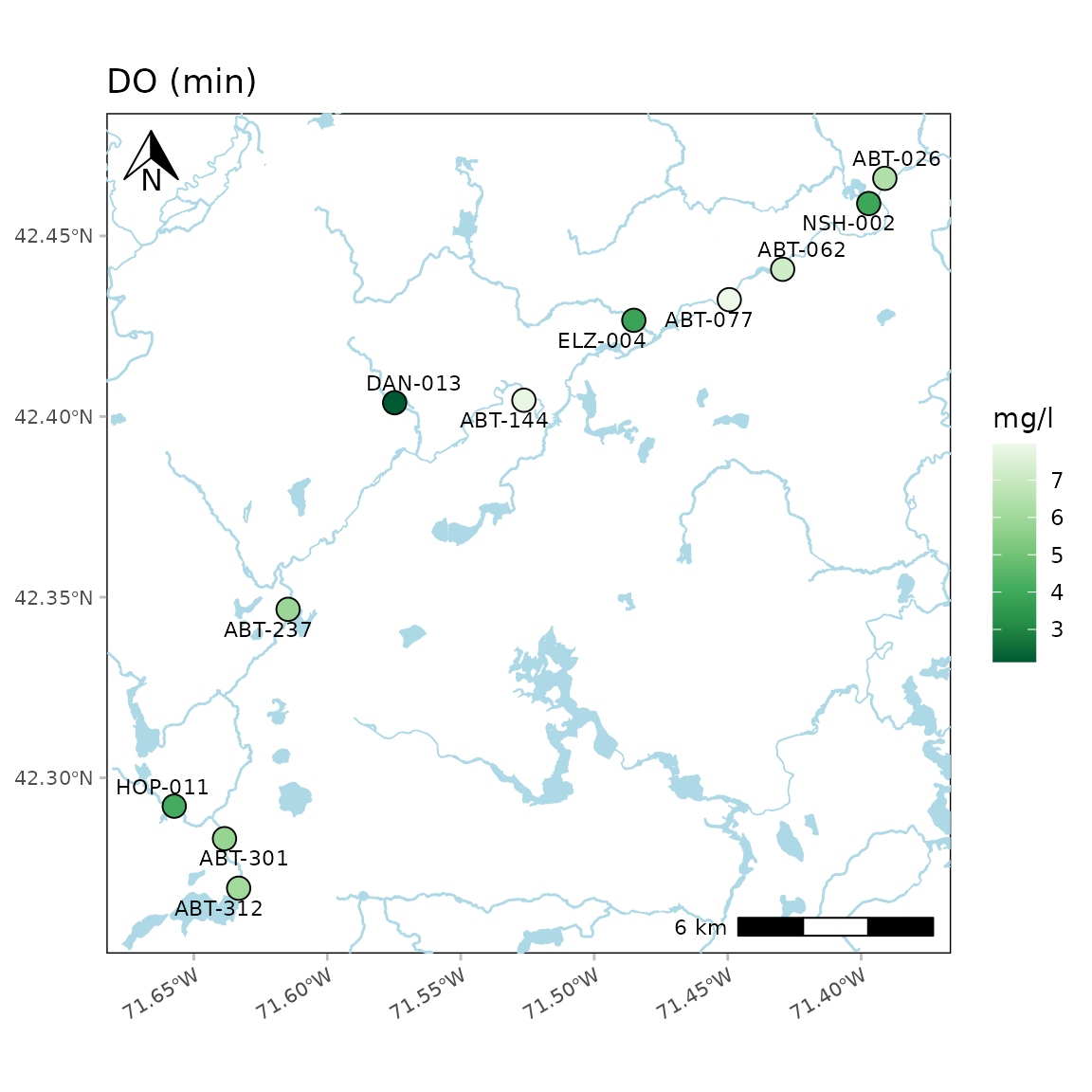By default, lines and polygons of natural water bodies defined using the National Hydrography Dataset (NHD) are plotted. Using addwater = "medium" (default) will plot water bodies at medium detail. The level of detail can be changed to low or high using addwater = "low" or addwater = "high", respectively. Use addwater = NULL to not show any water features.

Water body detail as low:

anlzMWRmap(res = resdat, param = "DO", acc = accdat, sit = sitdat, addwater = "low", warn = F)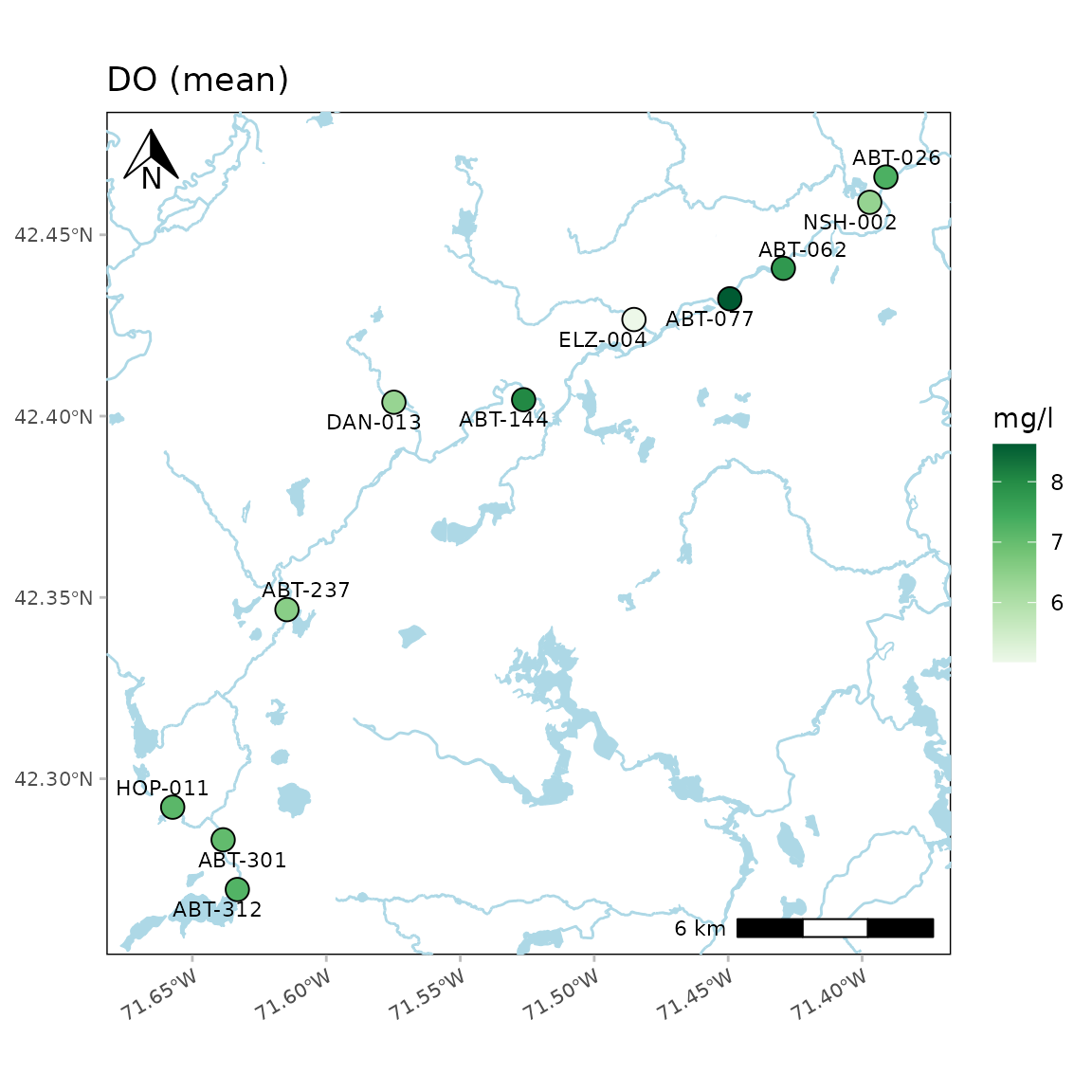Water body detail as high:

anlzMWRmap(res = resdat, param = "DO", acc = accdat, sit = sitdat, addwater = "high", warn = F)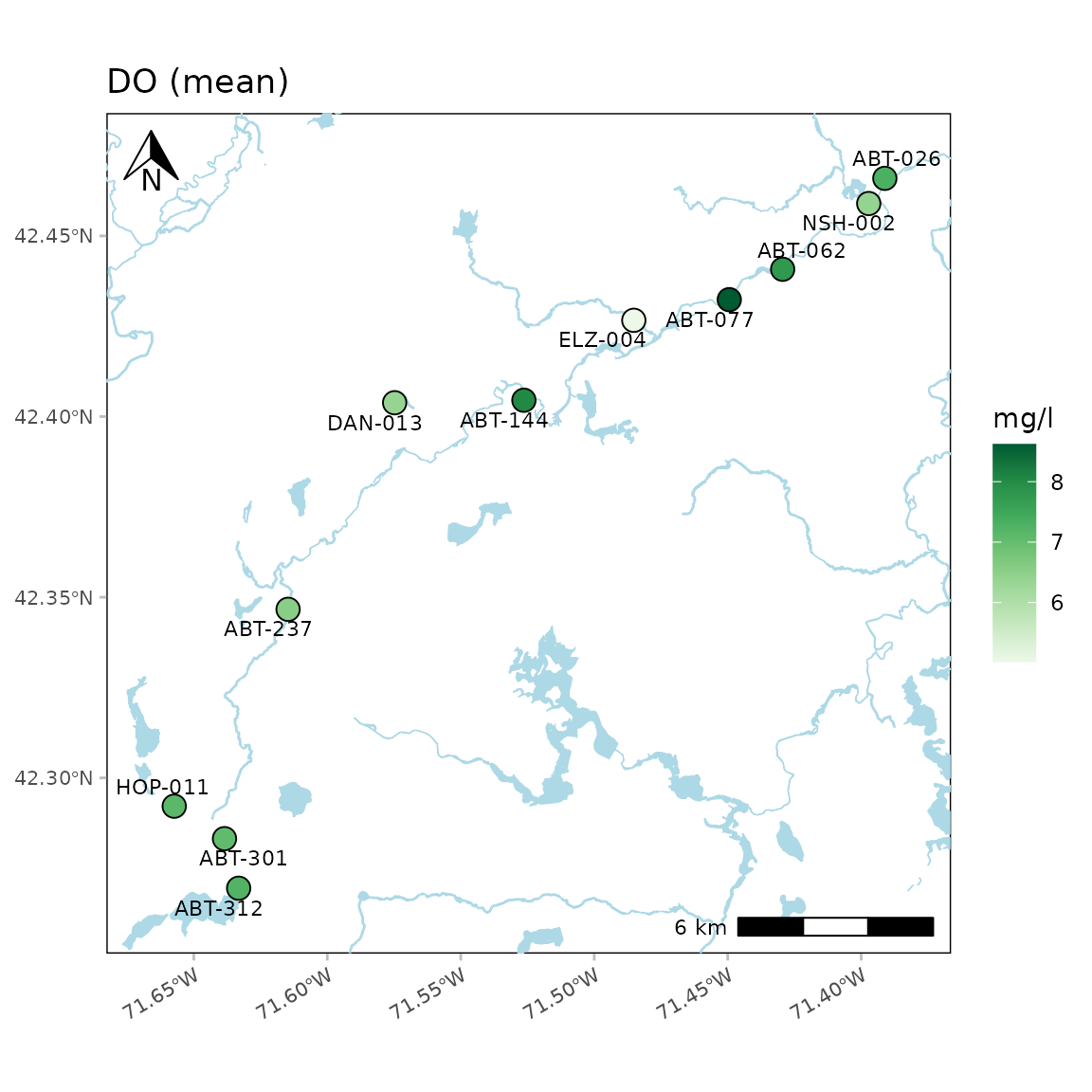A north arrow, scale bar, and labels are also plotted. These can be suppressed by setting the appropriate arguments to NULL.

anlzMWRmap(res = resdat, param = "DO", acc = accdat, sit = sitdat, northloc = NULL, scaleloc = NULL, labsize = NULL, warn = F)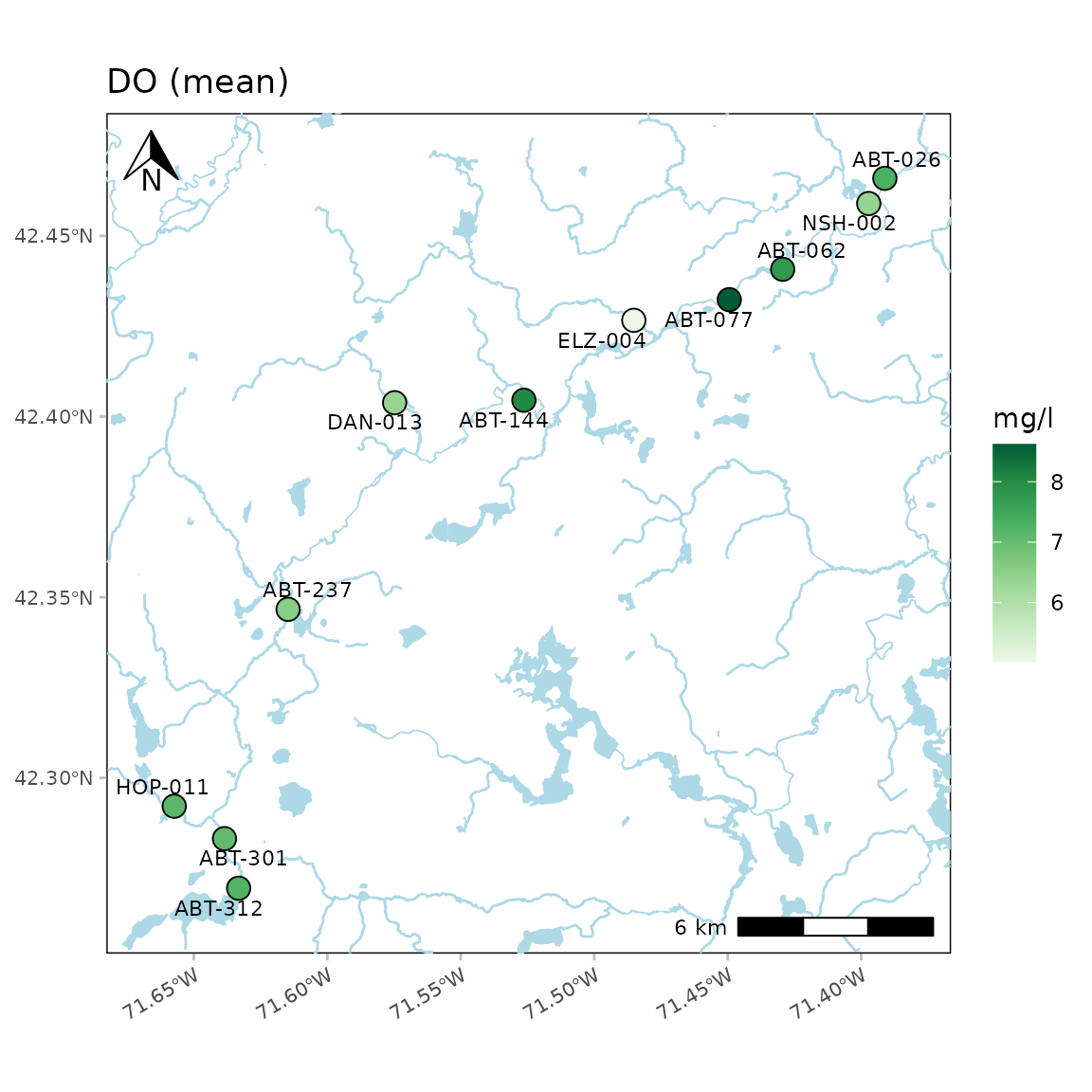Locations of the north arrow and scale bar can be changed with the same arguments by specifying "tl", "tr", "bl", or "br" for top-left, top-right, bottom-left, or bottom-right.

anlzMWRmap(res = resdat, param = "DO", acc = accdat, sit = sitdat, northloc = "br", scaleloc = "tl", warn = F)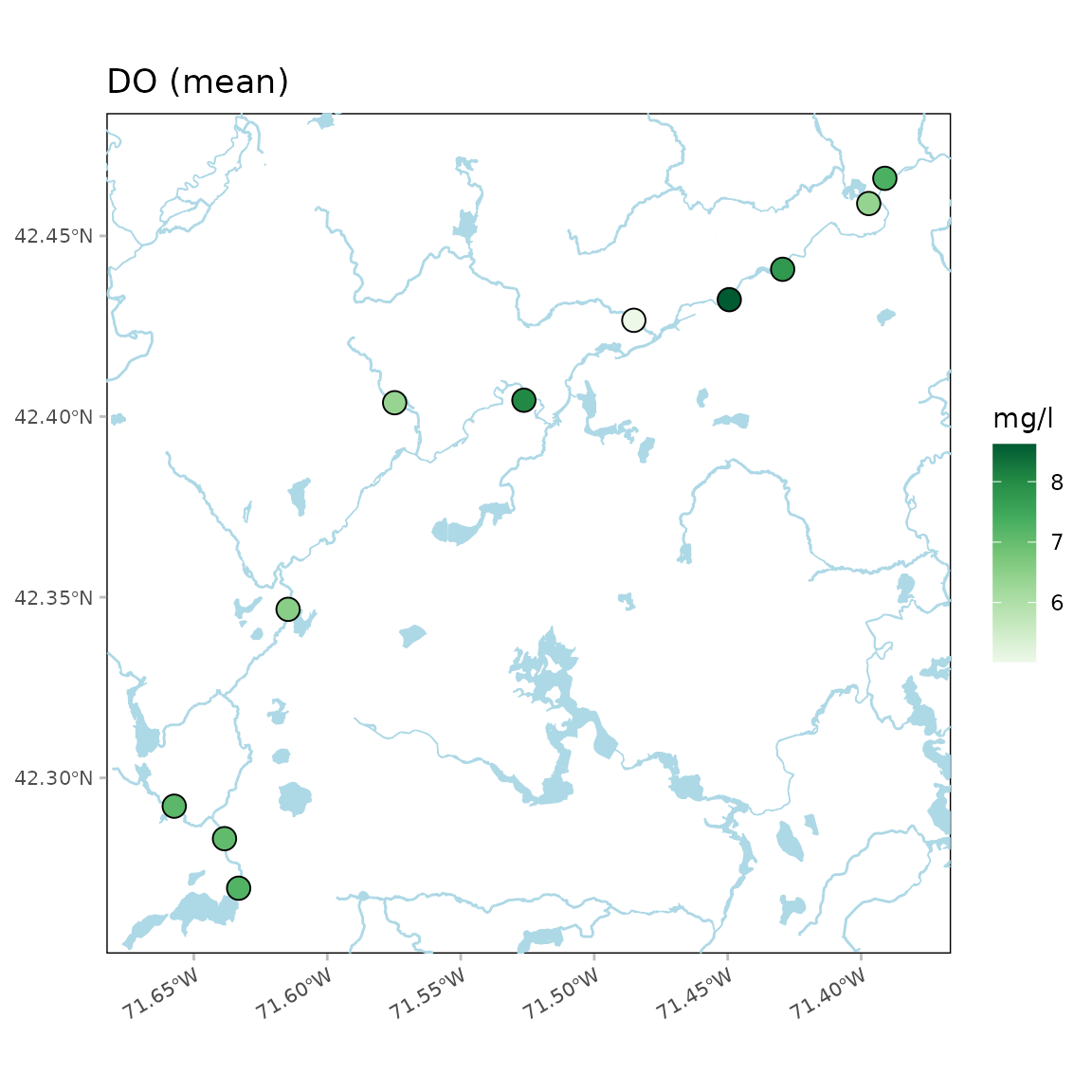The latitude and longitude text on the plot axes can be suppressed using latlon = FALSE.

anlzMWRmap(res = resdat, param = "DO", acc = accdat, sit = sitdat, latlon = F, warn = F)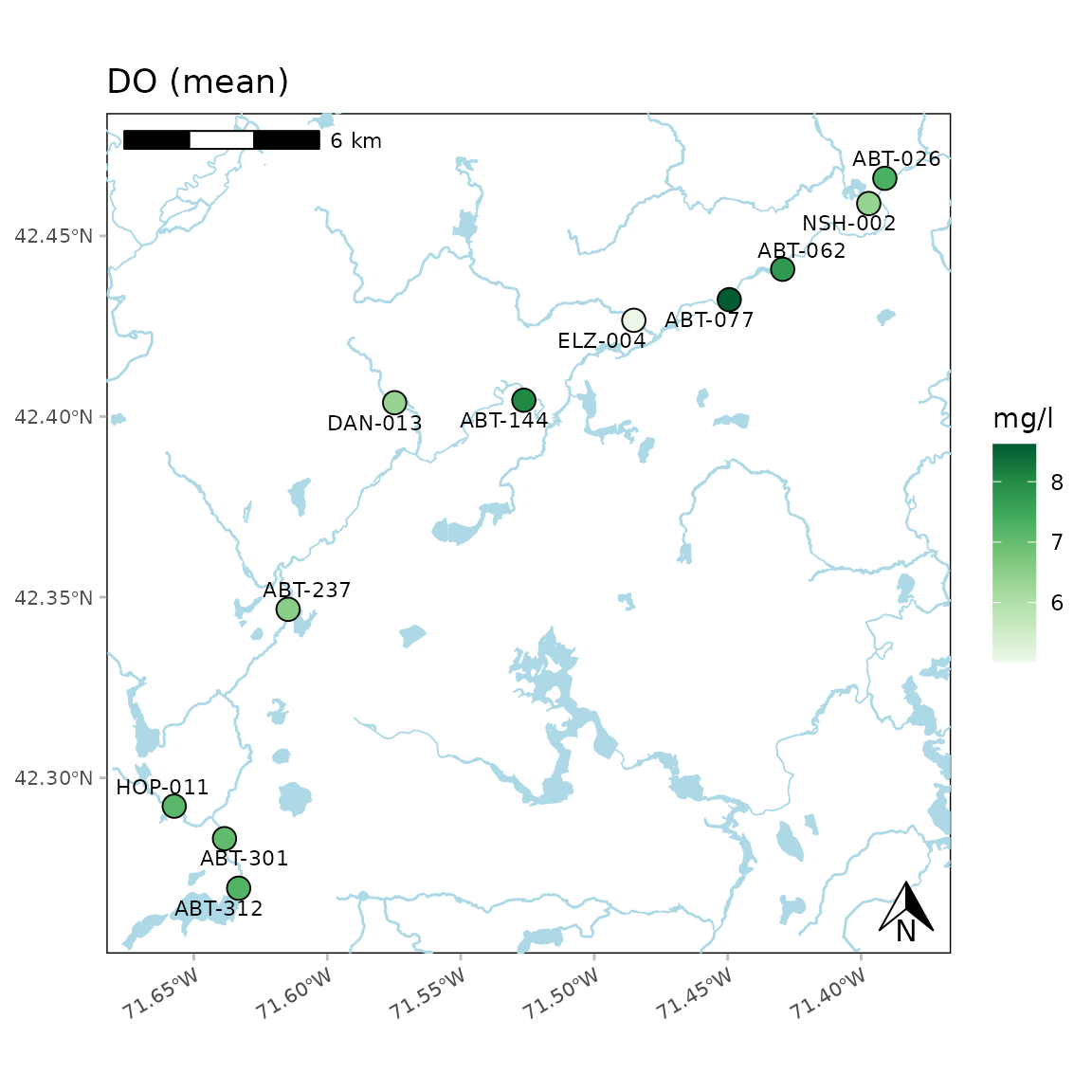The color palette for the average values can also be changed. Any palette from RColorBrewer can be used. These could include any of the sequential color palettes, e.g., "Greens", "Blues", etc. The diverging and qualitative palettes will also work, but may return uninterpretable color scales. In the following example, the diverging "Spectral" palette is used.

anlzMWRmap(res = resdat, param = "DO", acc = accdat, sit = sitdat, palcol = "Spectral", warn = F)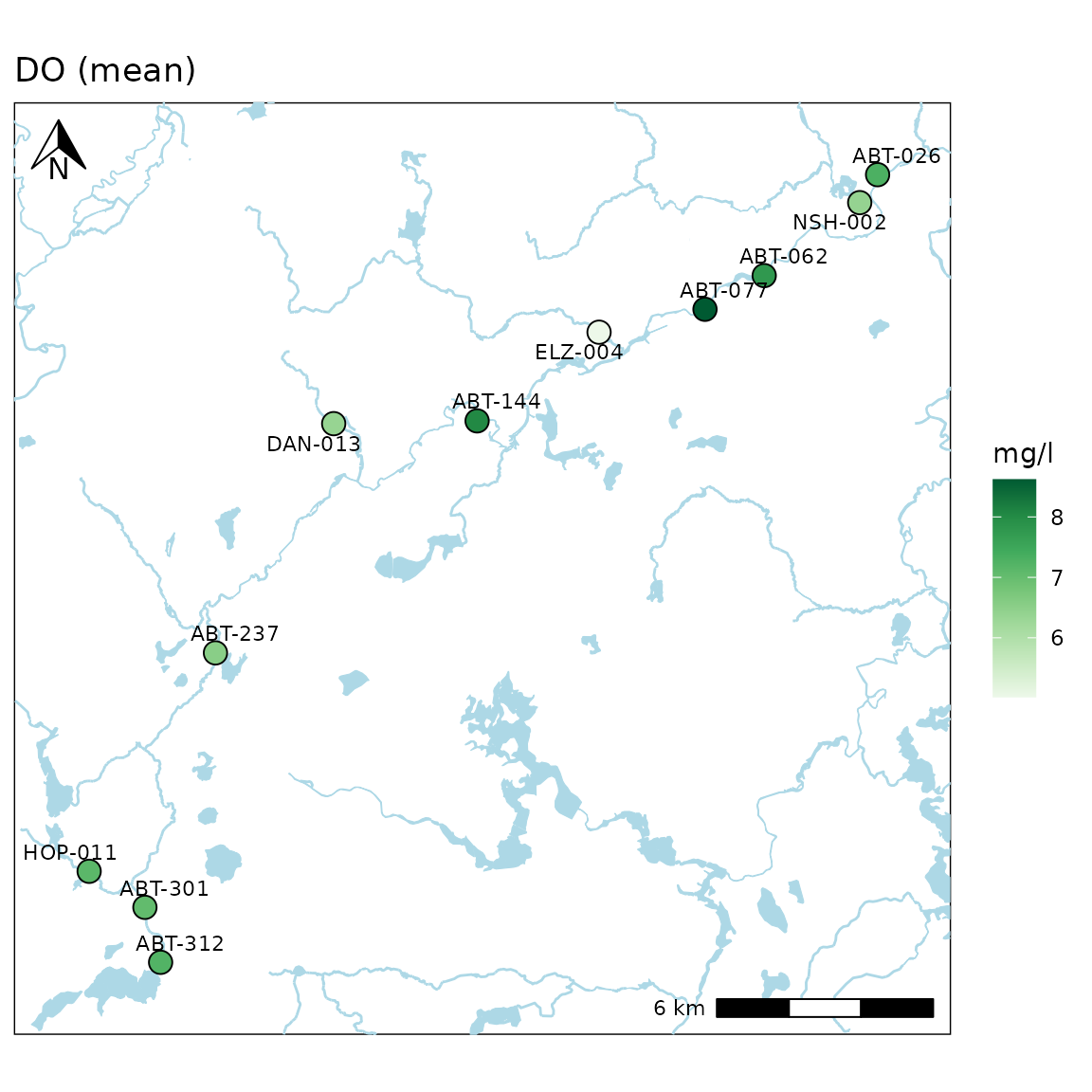The buffered distance around the points can be increased using the buffdist argument (in kilometers).

anlzMWRmap(res = resdat, param = "DO", acc = accdat, sit = sitdat, buffdist = 20, warn = F)A base map can be included as well using the maptype argument. Options include any stamen map as "terrain", "terrain-background" (default), "terrain-labels", "terrain-lines", "toner", "toner-2010", "toner-2011", "toner-background", "toner-hybrid", "toner-labels", "toner-lines", "toner-lite", or "watercolor". The zoom argument can be helpful when using a basemap. The default zoom argument is set to 11 and decreasing the number will download a base map with lower resolution. This can decrease map processing times for large areas.

A "terrain" base map:

anlzMWRmap(res = resdat, param = "DO", acc = accdat, sit = sitdat, maptype = "terrain", warn = F, addwater = NULL)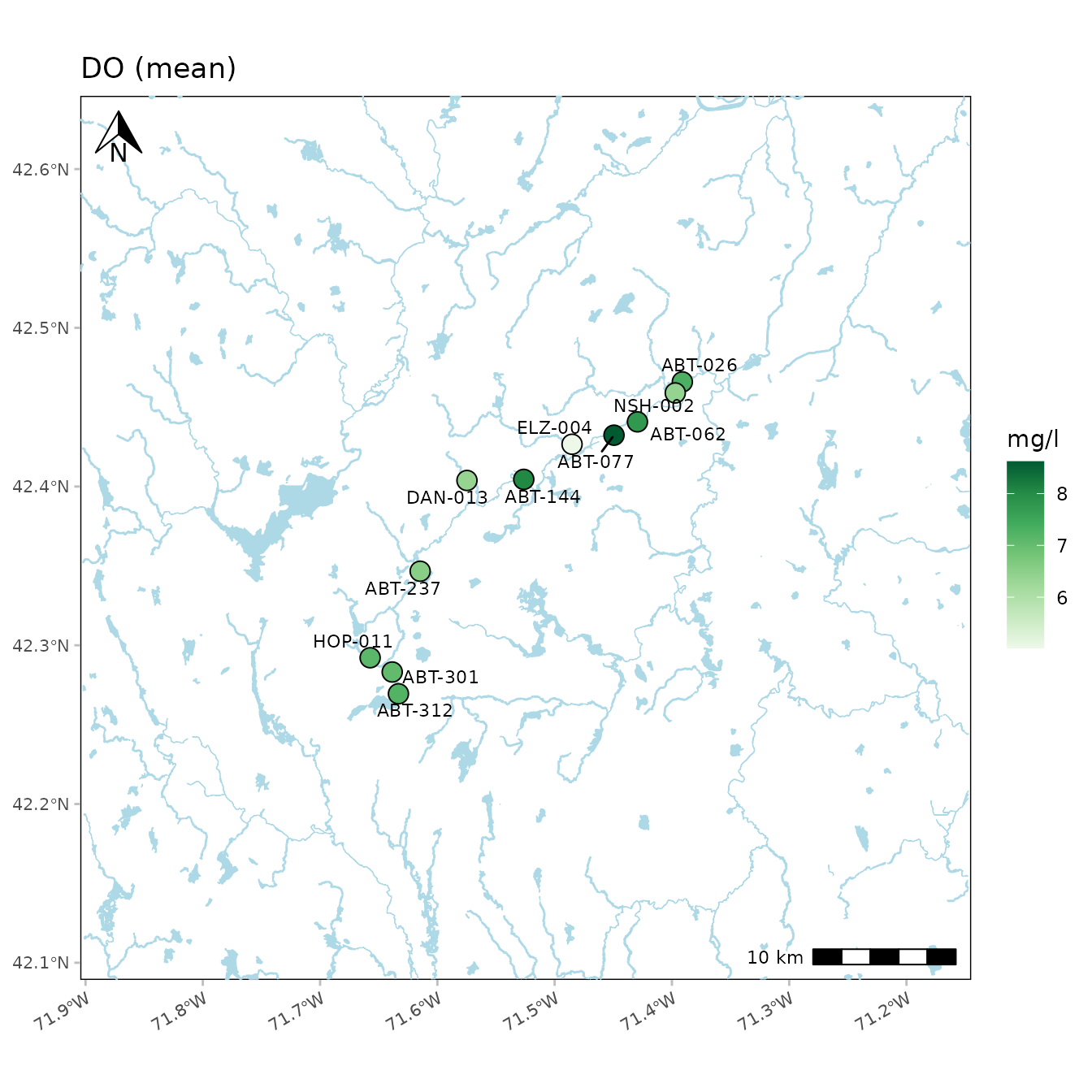A "terrain-lines" base map:

anlzMWRmap(res = resdat, param = "DO", acc = accdat, sit = sitdat, maptype = "terrain-lines", warn = F, addwater = NULL)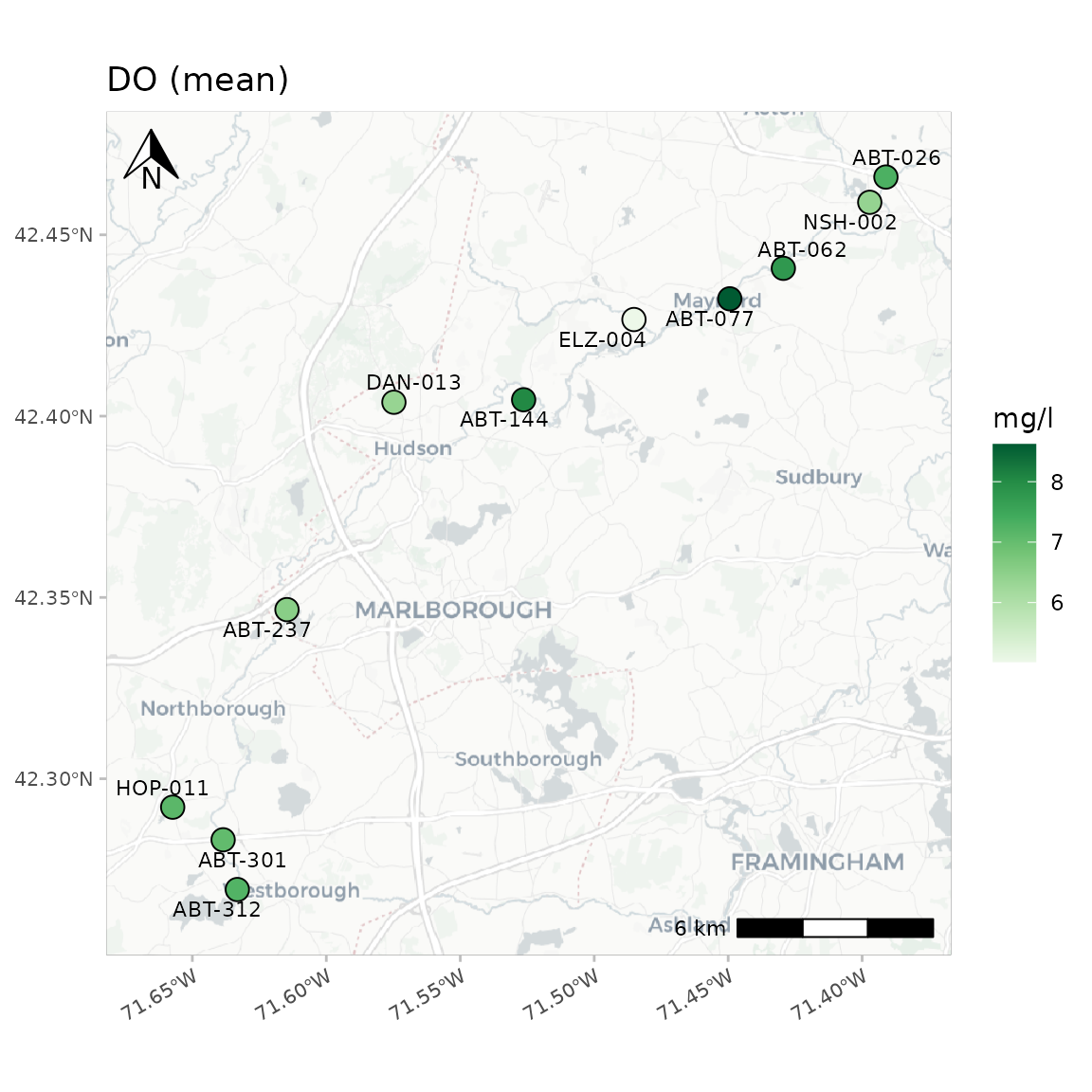A "toner" base map:

anlzMWRmap(res = resdat, param = "DO", acc = accdat, sit = sitdat, maptype = "toner", warn = F, addwater = NULL)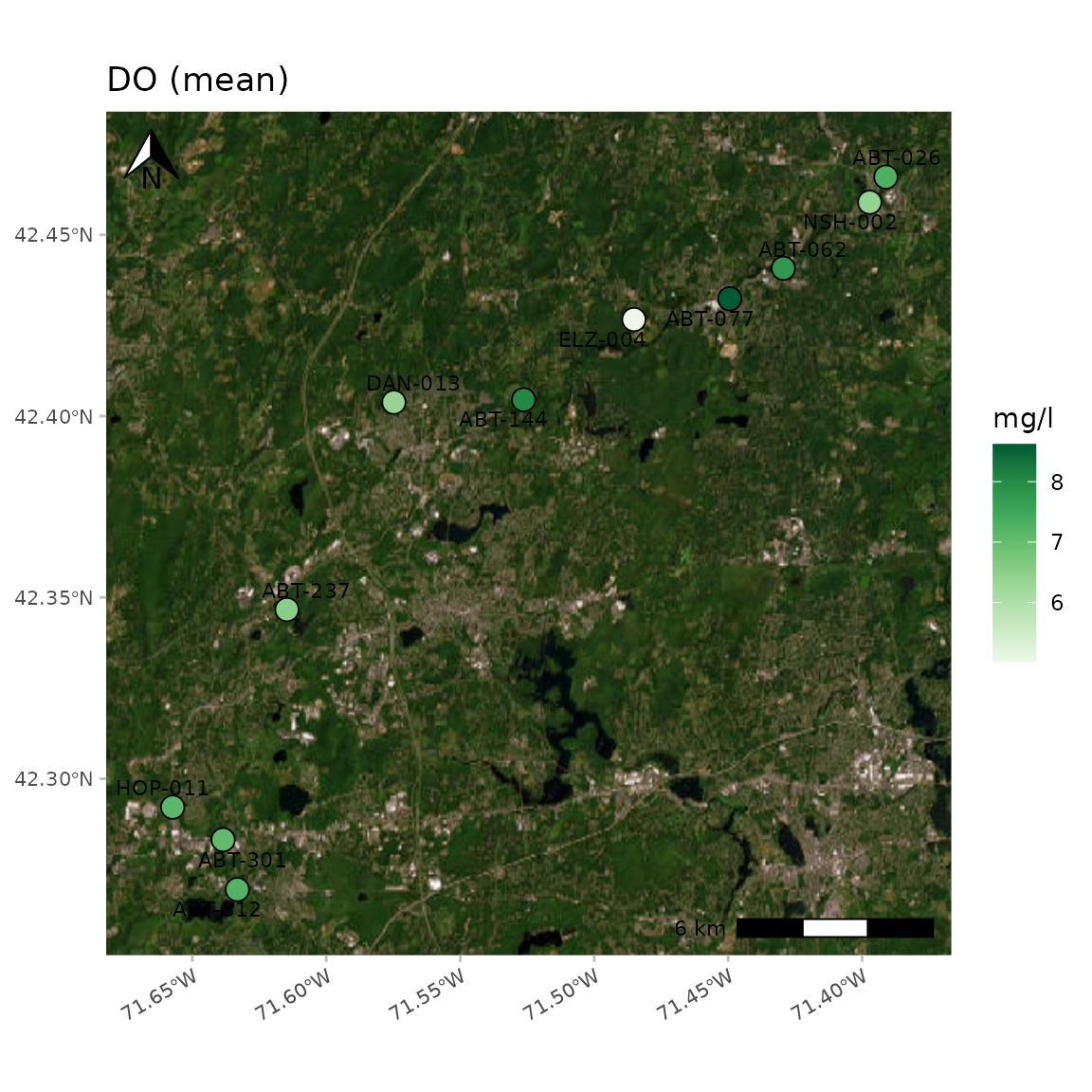A "toner-labels" base map:

anlzMWRmap(res = resdat, param = "DO", acc = accdat, sit = sitdat, maptype = "toner-labels", warn = F, addwater = NULL)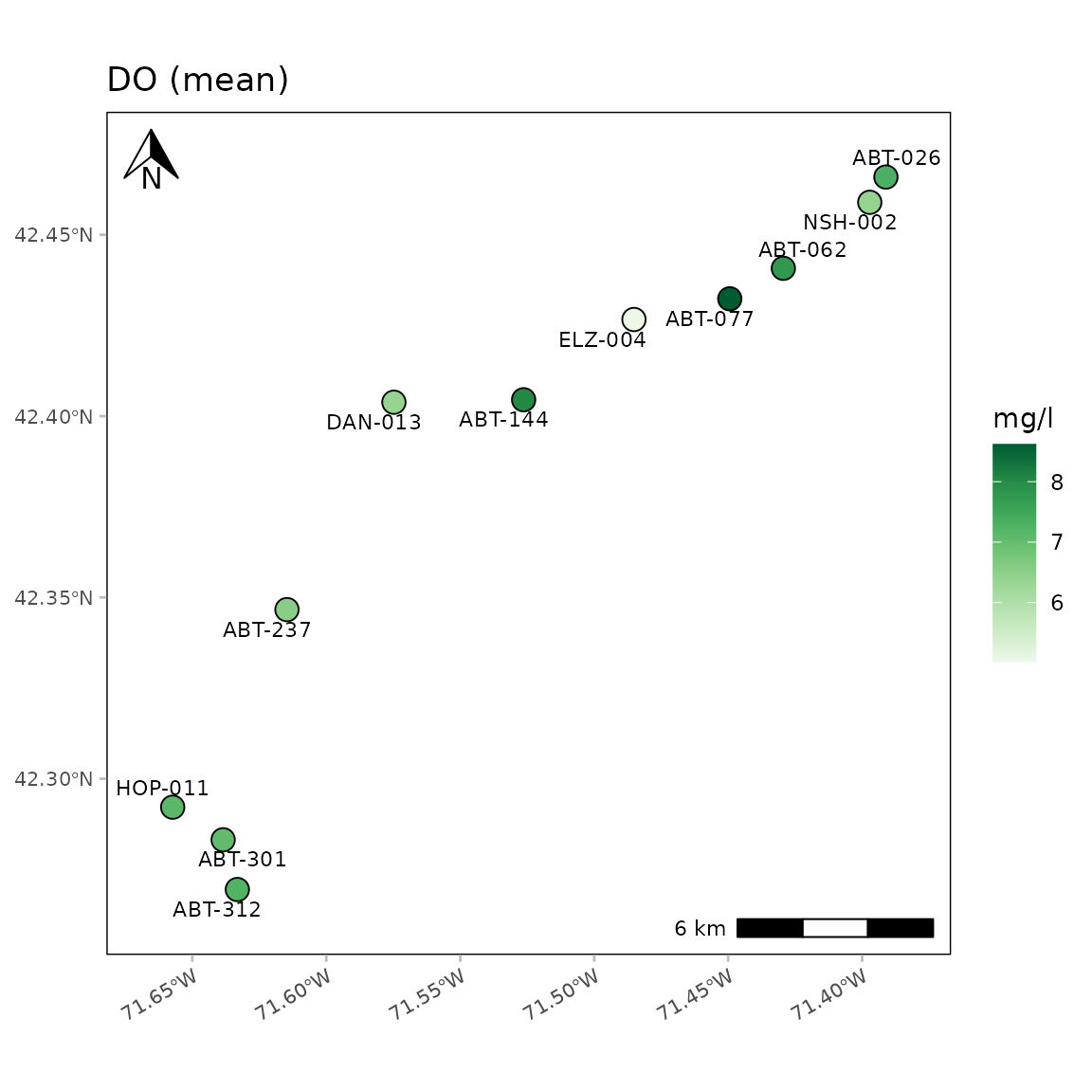A "watercolor" base map:

anlzMWRmap(res = resdat, param = "DO", acc = accdat, sit = sitdat, maptype = "watercolor", warn = F, addwater = NULL)A map with no base map or water bodies:

anlzMWRmap(res = resdat, param = "DO", acc = accdat, sit = sitdat, maptype = NULL, warn = F, addwater = NULL)